# Using linear programming to assess spatial access

So one of the problems I have been thinking about at work is assessing spatial access to providers. Some common metrics are ‘distance to nearest’, or combining distance as well as total provider capacity into one metric, the two-step floating catchment method (2SFCA).

So imagine we are trying to evaluate the coverage of opioid treatment facilities relative to the spatial distribution of people abusing opioids. Say for example we have two areas, A and B. Area A has a facility that can treat 50 people, but has a total of 100 people who need service. Now Area B has no treatment provider, but only has 10 people that need service.

If you looked at distance to nearest provider, location B would look worse than A. So you may think to yourself – we should open up a facility in Area B. But if you look at the total number of people, as well as the capacity of the treatment provider in Area A, it would probably be a better investment to expand the capacity of treatment center A. It has more potential demand.

The 2SFCA partially captures this, but I am going to show a linear programming approach that can decompose the added benefit of adding capacity to a particular provider, or adding in potential new providers. I have the posted these functions on github, but can walk through the linear programming model, and how to assess potential policy interventions in that framework.

So first lets make just a simple set of data, we have 10 people with XY coordinates, and 2 service providers. The two service providers have capacity 3 and 5, so we cannot cover 2 of the people.

``````# Python example code
import numpy as np
import pandas as pd
import pulp

# locations of people
id = range(10)
px = [1,1,3,3,4,5,6,6,9,9]
py = [2,8,1,8,4,6,1,2,5,8]
peop = pd.DataFrame(zip(id,px,py),columns=['id','px','py'])

# locations of 2 providers & capacity 3/5
hid = [1,2]
hx = [1,8]
hy = [1,8]
hc = [3,5] # so cant cover 2 people
prov = pd.DataFrame(zip(hid,hx,hy,hc),columns=['hid','hx','hy','hc'])``````

Now for the subsequent model I show, it is going to assign the people to the nearest possible provider, given the distance constraints. To make the model feasible, we need to add in a slack provider that can soak up the remaining people not covered. We set this location to somewhere very far away, so the model will only assign people to this slack provider in the case no other options are available.

``````# add in a slack location to the providers
# very out of the way and large capacity
prov_slack = prov.iloc[,:].copy()
prov_slack['hid'] = 999
prov_slack['hx'] = 999
prov_slack['hy'] = 999
prov_slack['hc'] = peop.shape

Now the decision model looks at all pairwise combinations between people and providers (potentially eliminating combinations that are too far away to be reasonable). So I do the cross join between the people/providers, and then calculate the Euclidean distance between them. I set the distance for the slack provider to a very high value (here 9999).

``````# distance between people/providers
peop['const'] = 1
cross['dist'] = np.sqrt( (cross['px']-cross['hx'])**2 +
(cross['py']-cross['hy'])**2 )
cross.set_index(['id','hid'],inplace=True)
# setting max distance for outside provider to 9999
cross.loc[cross.xs(999,level="hid",drop_level=False).index,"dist"] = 9999``````

Now we are ready to fit our linear programming model. First our objective is to minimize distances. (If all inputs are integers, you can use continuous decision variables and it will still return integer solutions.)

``````# now setting up linear program
# each person assigned to a location
# locations have capacity
# minimize distance traveled

# Minimize distances
P = pulp.LpProblem("MinDist",pulp.LpMinimize)

# each pair gets a decision variable
D = pulp.LpVariable.dicts("DA",cross.index.tolist(),
lowBound=0, upBound=1, cat=pulp.LpContinuous)

# Objective function based on distance
P += pulp.lpSum(D[i]*cross.loc[i,'dist'] for i in cross.index)``````

And we have two types of constraints, one is that each person is assigned a single provider in the end:

``````# Each person assigned to a single provider
for p in peop['id']:
provl = cross.xs(p,0,drop_level=False).index
P += pulp.lpSum(D[i] for i in provl) == 1, f"pers_{p}"``````

As a note later on, I will expand this model to include multiple people from a single source (e.g. count of people in a zipcode). For that expanded model, this constraint turns into `pulp.lpSum(...) == tot` where `tot` is the total people in a single area.

The second constraint is that providers have a capacity limit.

``````# Each provider capacity constraint
peopl = cross.xs(h,level=1,drop_level=False)
pid = peopl.index
cap = peopl['hc'].iloc # should be a constant
P += pulp.lpSum(D[i] for i in pid) <= cap, f"prov_{h}"``````

Now we can solve the model, and look at who was assigned to where:

``````# Solve the model
P.solve(pulp.PULP_CBC_CMD())
# CPLEX or CBC only ones I know of that return shadow
pulp.value(P.objective) #print objective 20024.33502494309

# Get the person distances
res_pick = []
for ph in cross.index:
res_pick.append(D[ph].varValue)

cross['picked'] = res_pick
cross[cross['picked'] > 0.99]``````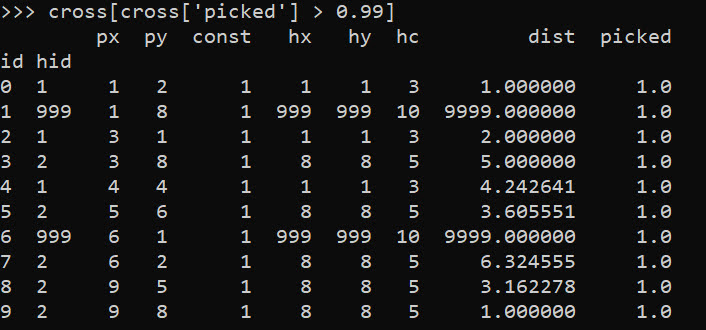So we can see two people were assigned the slack provider `999`. Note that some people are not even assigned the closest – person 7 `(6,2)` is assigned to provider `2` at a distance of `6.3`, it is only a distance of `5.1` away from provider 1. Because provider 1 has limited capacity though, they are assigned to provider 2 in the end.

In this framework, we can get the shadow price for the constraints, which says if we relax the constraint, how much it will improve our objective value.So if we add 1 capacity to provider 1, we will improve our objective by `-9994`.

``````# Get the shadow constraints per provider
o = [{'name':name, 'shadow price':c.pi, 'slack': c.slack}
for name, c in P.constraints.items()]
sc = pd.DataFrame(o)
print(sc)``````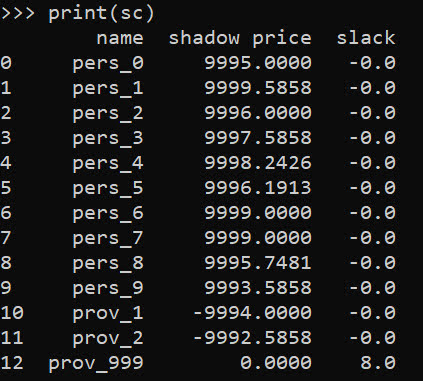I have helper functions at the github link above, so I don’t need to go through all of these motions again. You input your people matrix and the field names for the `id, x, y, totn` values.

And then you input your provider matrix with the field names for the `providerid, x, y, prov_capacity` (note this is not the matrix with the additional slack provider, my code adds that in automatically). The final two arguments limit the potential locations (e.g. here saying can’t assign a person to a provider over 12 distance away). And the last argument sets the super high distance penalty to people are not assigned.

``````# load in model functions
from assign_funcs import ProvAssign

# Const=1 is the total people per area
m1 = ProvAssign(peop,
['id','px','py','const'],
prov,
['hid','hx','hy','hc'],
12,
9999)

m1.solve(pulp.PULP_CBC_CMD())
# see the same objective as before``````

Now we can go ahead and up our capacity at provider 1 by 1, and see how the objective is reduced by -9994:

``````# if we up the provider capacity for
# prov by 1, the model objective goes
# down by -9994
prov['hc'] = [4,5]

m2 = ProvAssign(peop,
['id','px','py','const'],
prov,
['hid','hx','hy','hc'],
12,
9999)
m2.solve(pulp.PULP_CBC_CMD())
m1.obj - m2.obj # 9994!``````

Like I said, I extended this to the scenario that you don’t have individual people, but have multiple counts of people in a spatial area. What can happen in this scenario is one source location can send people to multiple providers. Here you can see that source location 4 (total of 12 people), 5 were sent to provider 1, and 7 were sent to provider 2.

``````# Can make these multiple people, 100 total
peop['tot'] = [10,15,5,10,12,11,20,6,9,2]
prov['cap'] = [40,50] # should assign 10 people to slack

m3 = ProvAssign(peop,
['id','px','py','tot'],
prov,
['hid','hx','hy','cap'],
12,
9999)
m3.solve(pulp.PULP_CBC_CMD())
# Can see the assignments from one
# source can spill over into multiple
# provider locations
m3.assign``````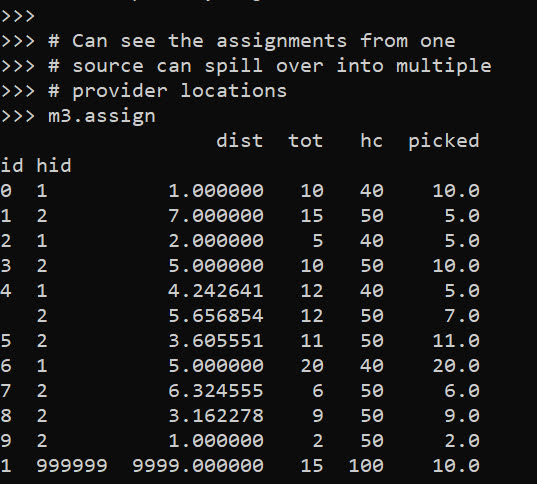So one of the things I like about this approach I already showed, we can do hypothetical scenarios ‘add capacity’ and see how it improves overall travel. Another potential intervention is to just place dummy 0 capacity providers over the study area, then look at the shadow constraints to see the best locations to open new facilities. Here I add in a potential provider at location `(5,5)`.

``````# Can add in hypothetical providers with
# no capacity (make new providers)
# and check out the shadow
p2 = prov_slack.copy()
p2['hid'] = 10
p2['hx'] = 5
p2['hy'] = 5
p2['hc'] = 0
p2['cap'] = 0

m4 = ProvAssign(peop,
['id','px','py','tot'],
['hid','hx','hy','cap'],
10,
9999)

# we now don't have source9 and prov1
m4.cross``````

Here in the code, I also have a function to limit potential assignments. Here if I set that limit to 10, it only just prevents id 9 `(9,8)` from being assigned to provider 1 `(1,1)`, which is a distance of 10.6 away. This is useful with larger datasets, in which you may not be able to fit all of the pairwise distances into memory and model. (Although this model is pretty simple, you may be able to look at +1 million pairwise combos and solve in a reasonable time with open source solvers.)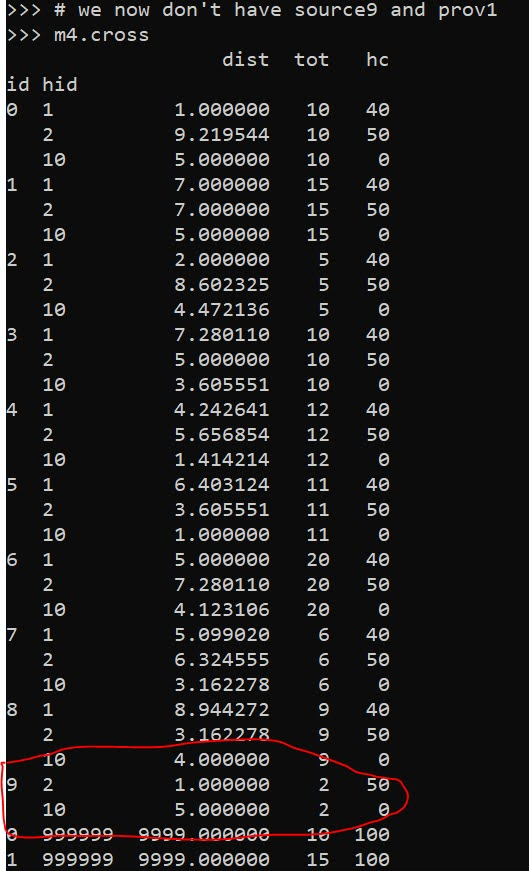Now we can go ahead and solve this model. I have a bunch of helper functions as well, so after solving we can check out the shadow price matrix:

``````# Solve m4 model
m4.solve(pulp.PULP_CBC_CMD())

# can see the new provider 10
# if we added capacity would
# decrease distance travelled by -9996.2426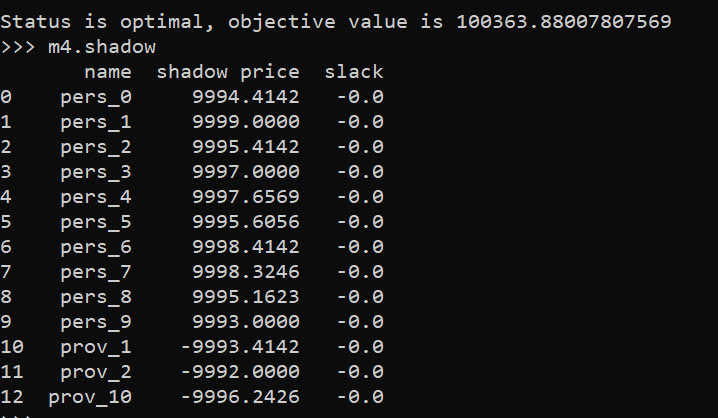And based on this, it does look like we will have the best improvement by adding capacity at our hypothetical new provider 10, as oppossed to adding capacity at provider 1 or 2. Lets see what happens if you add 10 capacity to our hypothetical provider:

``````# Now lets add capacity for new provider
# by 10, should the objective go down
# by 10*-9996.2426 ?

m5 = ProvAssign(peop,
['id','px','py','tot'],
['hid','hx','hy','cap'],
12,
9999)
m5.solve(pulp.PULP_CBC_CMD())

# Not quite -9996.2 but close!
(m5.obj - m4.obj)/10``````

We can see that the objective was not quite reduced by the expected, but is close. If it is not feasible to add a totally new provider, but simpler to give resources to expand current ones, we can see what will happen if we expand provider 1 by 10.

``````# we could add capacity
# to provider 1 by 10
# as well

m6 = ProvAssign(peop,
['id','px','py','tot'],
['hid','hx','hy','cap'],
10,
9999)
m6.solve(pulp.PULP_CBC_CMD())

# Not quite -9993.4 but close!
(m6.obj - m4.obj)/10``````

In addition to doing different policy interventions in this approach, I provide different metrics to assess distance traveled and coverage. So going back to model 4, we can look at which areas have limited coverage. It only ends up that source area 1 has 10/15 people not covered. The rest of the areas are covered.

``````# Can look at sources and see stats
# for distance travelled as well
# as potential non-coverage
m4.source_stats``````The fields are `Trav` is the average distance travelled for people who are covered (so could have high coverage but those people have to go a ways). `Tot` is the total number of people within that particular source area, and picked/not-covered are those assigned a provider/not assigned a provider (so go to the slack) respectively.

I additionally I have stats available rolled up to providers, mostly based on the not covered nearby. One way that the shadow doesn’t work, if you only have 10 people nearby, it doesn’t make sense to expand capacity to any more than 10 people. Here you can see the shadow price, as well as those not covered that are nearby to that provider.

``````# Can look at providers
# and see which ones would result in
# most reduced travel given increased coverage
# Want high NotCover and low shadow price
m4.prov_stats``````The other fields, `hc` is the listed capacity. `Trav` is the total travel for those not covered. The last field `bisqw`, is a way to only partially count not covered based on distance. It uses the bi-square kernel weight, based on the max distance field you provided in the model object. So if many people are nearby it will get a higher weight.

Here good add capacity locations are those with low shadow prices, and high notcovered/bisqw fields.

Note these won’t per se give the best potential places to add capacity. It may be the best new solution is to spread out new capacity – so here instead of adding 10 to a single provider, add a few to different providers.

You could technically figure that out by slicing out those leftover people in the `m4.source_stats` that have some not covered, adding in extra capacity to each provider, and then rerunning the algorithm and seeing where they end up.

Again I like this approach as it lets you articulate different policy proposals and see how that changes the coverage/distance travelled. Although no doubt there are other ways to formulate the problem that may make sense, such as maximizing coverage or making a coverage/distance bi-objective function.

# Optimal and Fair Spatial Siting

A bit of a belated MLK day post. Much of the popular news on predictive or machine learning algorithms has a negative connotation, often that they are racially biased. I tend to think about algorithms though in almost the exact opposite way – we can adjust them to suit our objectives. We just need to articulate what exactly we mean by fair. This goes for predictive policing (Circo & Wheeler, 2021; Liberatore et al., 2021; Mohler et al., 2018; Wheeler, 2020) as much as it does for any application.

I have been reading a bit about spatial fairness in siting health resources recently, one example is the Urban Institutes Equity Data tool. For this tool, you put in where your resources are currently located, and it tells you whether those locations are located in areas that have demographic breakdowns like the overall city. So this uses the container approach (not distance to the resources), which distance traveled to resources is probably a more typical way to evaluate fair spatial access to resources (Hassler & Ceccato, 2021; Koschinsky et al., 2021).

Here what I am going to show is instead of ex-ante saying whether the siting of resources is fair, I construct an integer linear program to site resources in a way we define to be fair. So imagine that we are siting 3 different locations to do rapid Covid testing around a city. Well, we do the typical optimization and minimize the distance traveled for everyone in the city on average to those 3 locations – on average 2 miles. But then we see that white people on average travel 1.9 miles, and minorities travel 2.2 miles. So it that does not seem so fair does it.

I created an integer linear program to take this difference into account, so instead of minimizing average distance, it minimizes:

``White_distance + Minority_distance + |White_distance - Minority_distance|``

So in our example above, if we had a solution that was white travel 2.1 and minority 2.1, this would be a lower objective value than (4.2), than the original minimize overall travel (1.9 + 2.2 + 0.3 = 4.4). So this gives each minority groups equal weight, as well as penalizes if one group (either whites or minorities) has much larger differences.

I am not going to go into all the details. I have python code that has the functions (it is very similar to my P-median model, Wheeler, 2018). The codes shows an example of siting 5 locations in Dallas (and uses census block group centroids for the demographic data). Here is a map of the results (it has points outside of the city, since block groups don’t perfectly line up with the city boundaries).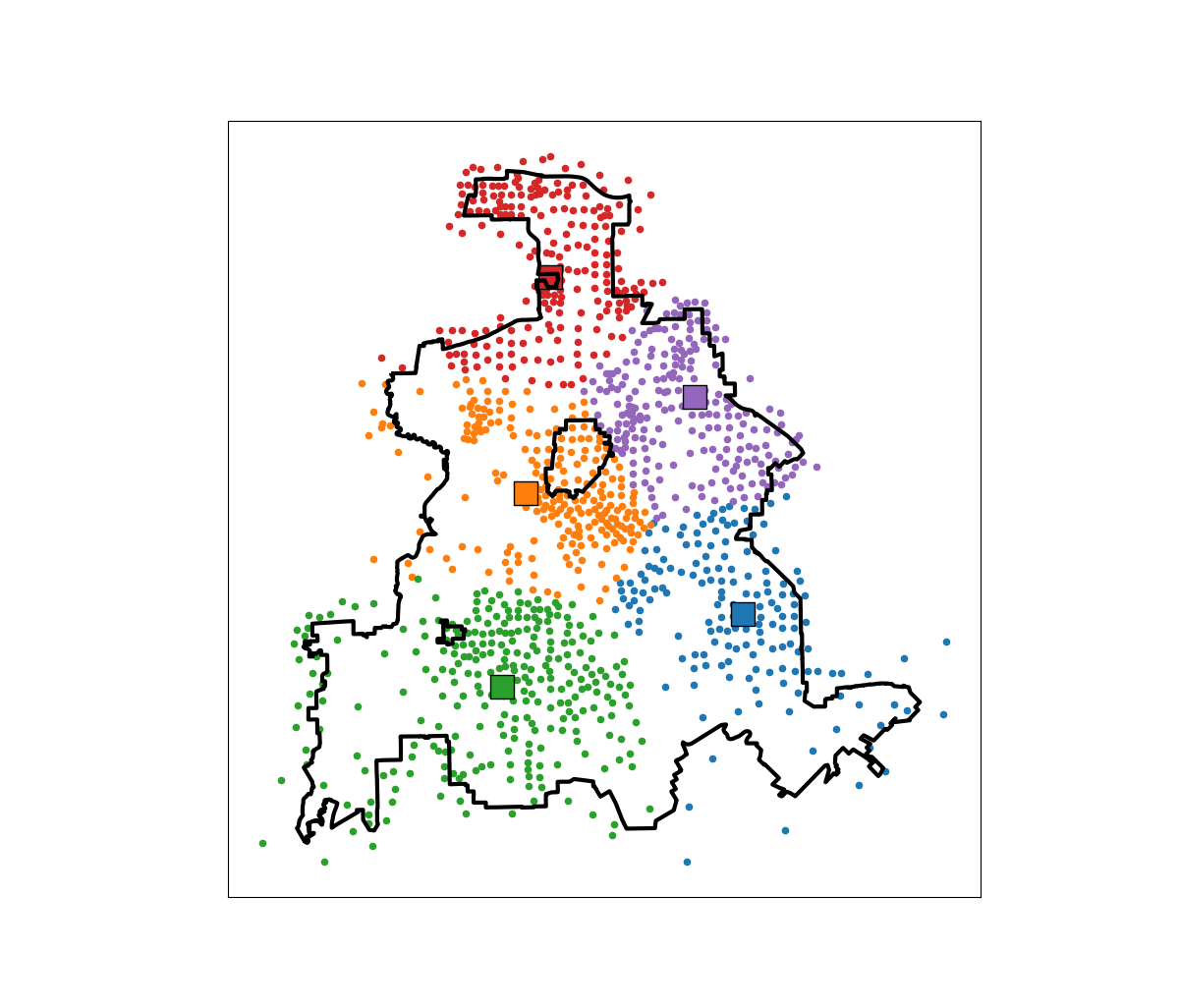In this example, if we choose 5 locations in the city to minimize the overall distance, the average travel is just shy of 3.5 miles. The average travel for white people (not including Hispanics) is 3.25 miles, and for minorities is 3.6 miles. When I use my fair algorithm, the white average distance is 3.5 miles, and the minority average distance is 3.6 miles (minority on average travels under 200 more feet on average than white).

So this is ultimately a trade off – it ends up pushing up the average distance a white person will travel, and only slightly pushes down the minority travel, to balance the overall distances between the two groups. It is often the case though that one can be somewhat more fair, but in only results in slight trade-offs though in the overall objective function (Rodolfa et al., 2021). So that trade off is probably worth it here.

# Generating multiple solutions for linear programs

I had a question the other day on my TURF analysis about generating not just the single best solution for a linear programming problem, but multiple solutions. This is one arena I like the way people typically do genetic algorithms, in that they have a leaderboard with multiple top solutions. Which is nice for exploratory data analysis.

This example though it is pretty easy to amend the linear program to return exact solutions by generating lazy constraints. (And because the program is fast, you might as well do it this way as well to get an exact answer.) The way it works here, we have decision variables for products selected. You run the linear program, and then collect the k products selected, then add a constraint to the model in the form of:

``Sum(Product_k) <= k-1 ``

Then rerun the model with this constraint, get the solution, and then repeat. This constraint makes it so the group of decision variables selected cannot be replicated (and in the forbidden set). Python’s pulp makes this quite easy, and here is a function to estimate the TURF model I showed in that prior blog post, but generate this multiple leaderboard:

``````import pandas as pd
import pulp

# Function to get solution from turf model
def get_solution(prod_dec,prod_ind):
'''
prod_dec - decision variables for products
prod_ind - indices for products
'''
picked = []
for j in prod_ind:
if prod_dec[j].varValue >= 0.99:
picked.append(j)
return picked

# Larger function to set up turf model
# And generate multiple solutions
def turf(data,k,solutions):
'''
data - dataframe with 0/1 selected items
k - integer for products selected
solutions - integer for number of potential solutions to return
'''
# Indices for Dec variables
Cust = data.index
Prod = list(data)
# Problem and Decision Variables
P = pulp.LpProblem("TURF", pulp.LpMaximize)
Cu = pulp.LpVariable.dicts("Customers Reached", [i for i in Cust], lowBound=0, upBound=1, cat=pulp.LpInteger)
Pr = pulp.LpVariable.dicts("Products Selected", [j for j in Prod], lowBound=0, upBound=1, cat=pulp.LpInteger)
# Objective Function (assumes weight = 1 for all rows)
P += pulp.lpSum(Cu[i] for i in Cust)
# Reached Constraint
for i in Cust:
#Get the products selected
p_sel = data.loc[i] == 1
sel_prod = list(p_sel.index[p_sel])
#Set the constraint
P += Cu[i] <= pulp.lpSum(Pr[j] for j in sel_prod)
# Total number of products selected constraint
P += pulp.lpSum(Pr[j] for j in Prod) == k
# Solve the equation multiple times, add constraints
res = []
km1 = k - 1
for _ in range(solutions):
P.solve()
pi = get_solution(Pr,Prod)
# Lazy constraint
P += pulp.lpSum(Pr[j] for j in pi) <= km1
pi.append(pulp.value(P.objective))
res.append(pi)
# Turn results into nice dataframe
cols = ['Prod' + str(i+1) for i in range(k)]
res_df = pd.DataFrame(res, columns=cols+['Reach'])
res_df['Reach'] = res_df['Reach'].astype(int)
return res_df``````

And now we can simply read in our data, turn it into binary 0/1, and then generate the multiple solutions.

``````#This is simulated data from XLStat
#https://help.xlstat.com/s/article/turf-analysis-in-excel-tutorial?language=en_US

#Replacing the likert scale data with 0/1
prod_bin = 1*(prod_data > 3)

# Get Top 10 solutions
top10 = turf(data=prod_bin,k=5,solutions=10)
print(top10)``````

Which generates the results:

``````>>> print(top10)
Prod1       Prod2       Prod3       Prod4       Prod5  Reach
0   Product 4  Product 10  Product 15  Product 21  Product 25    129
1   Product 3  Product 15  Product 16  Product 25  Product 26    129
2   Product 4  Product 14  Product 15  Product 16  Product 26    129
3  Product 14  Product 15  Product 16  Product 23  Product 26    129
4   Product 3  Product 15  Product 21  Product 25  Product 26    129
5  Product 10  Product 15  Product 21  Product 23  Product 25    129
6   Product 4  Product 10  Product 15  Product 16  Product 27    129
7  Product 10  Product 15  Product 16  Product 23  Product 27    129
8   Product 3  Product 10  Product 15  Product 21  Product 25    128
9   Product 4  Product 10  Product 15  Product 21  Product 27    128``````

I haven’t checked too closely, but this looks to me offhand like the same top 8 solutions (with a reach of 129) as the XLStat website. The last two rows are different, likely because there are further ties.

For TURF analysis, you may actually consider a lazy constraint in the form of:

``Product_k = 0 for each k``

This is more restrictive, but if you have a very large pool of products, will ensure that each set of products selected are entirely different (so a unique set of 5 products for every row). Or you may consider a constraint in terms of customers reached instead of on products, so if solution 1 reaches 129, you filter those rows out and only count newly reached people in subsequent solutions. This ensures each row of the leaderboard above reaches different people than the prior rows.

# Solving the P-Median model

Wendy Jang, my former student at UTD and now Data Scientist for the Stanislaus County Sheriff’s Dept. writes in:

Hello Andy,

I have some questions about your posting in GitHub. Honestly, I am not good at Python at all. I was playing with your python code and trying to understand the work flow. Then, I can pretty much mimic what you did; however, I encountered some errors along the way.

I was able to follow through lines but then I am stuck on PMed function. Do you know what possibly caused this error? See the screenshot below.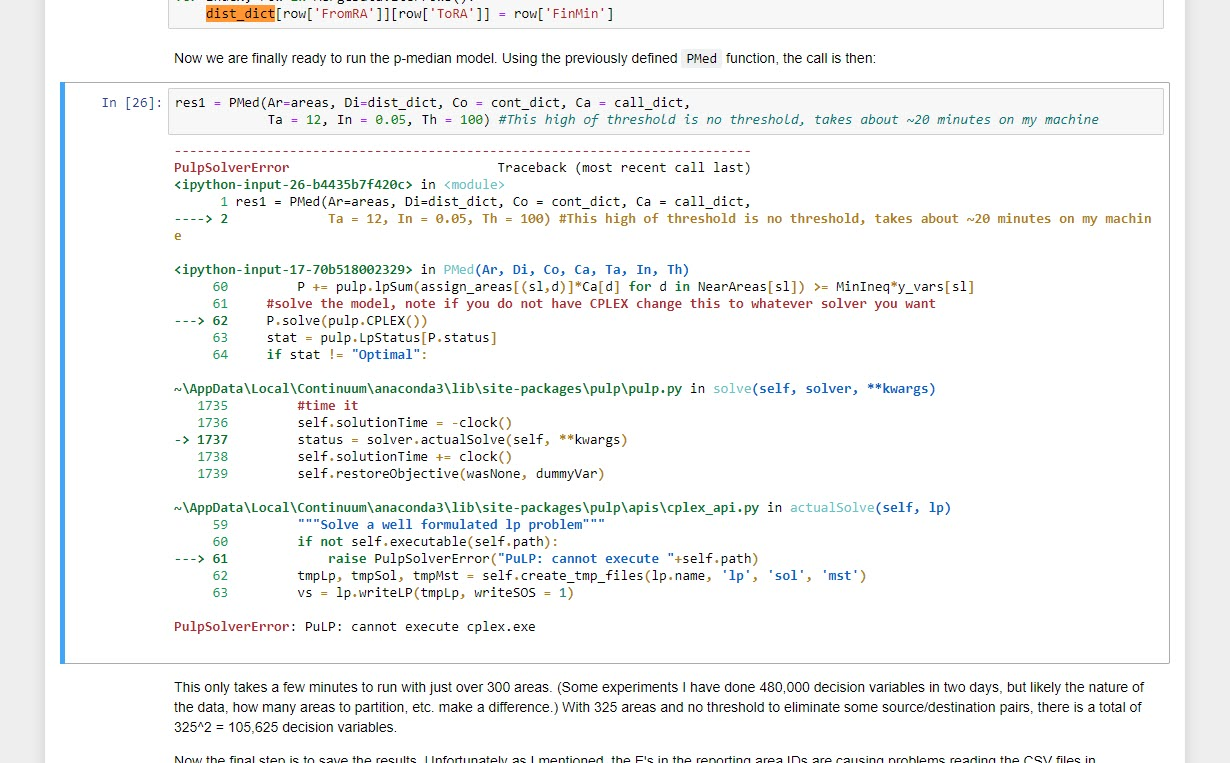Please advise. Thanks!

Specifically, Wendy is asking about my patrol redistricting example with workload inequality constraints. Wendy’s problem here is specifically she likely does not have CPLEX installed. CPLEX is free for academics, but unfortunately costs a bit of money for anyone else (not sure if they have cheaper licensing for public sector, looks like currently about \$200 a month).

This was a good opportunity to update some of my code, so now see the two `.py` files in the DataCreated folder, in particular `01_pmed_class.py` has a nice class function. I have additionally added in functionality to create a map, and more importantly eliminate subtours (my solution can potentially return disconnected areas). I have also added the ability to use different solvers.

For this problem CPLEX just works much better (takes around 10 minutes), but I was able to get the SCIP solver to return the same solution in around 5 hours. GLPK did not return a solution even letting it churn out for over 12 hours. I tested CBC for shorter time periods, but that appears to be a no-go as well.

So just a brief overview, the libraries I will be using (check out the end of the post for setting up the conda environment):

``````import pickle
import pulp
import networkx
import pandas as pd
from datetime import datetime
import matplotlib.pyplot as plt
import geopandas as gpd

class pmed():
"""
Ar - list of areas in model
Di - distance dictionary organized Di[a1][a2] gives the distance between areas a1 and a2
Co - contiguity dictionary organized Co[a1] gives all the contiguous neighbors of a1 in a list
Ca - dictionary of total number of calls, Ca[a1] gives calls in area a1
Ta - integer number of areas to create
In - float inequality constraint
Th - float distance threshold to make a decision variables
"""
def __init__(self,Ar,Di,Co,Ca,Ta,In,Th):``````

I do not copy-paste the entire `pmed` class in the blog post. It is long, but is the rewrite of my model from the paper. Next, to load in the objects I need to fit the model (which were created/pickled in the `00_PrepareData.py` file).

``````# Loading in data
data_loc = r'D:\Dropbox\Dropbox\PublicCode_Git\PatrolRedistrict\PatrolRedistrict\DataCreated\fin_obj.pkl'
areas, dist_dict, cont_dict, call_dict, nid_invmap, MergeData = pickle.load(open(data_loc,"rb"))

# shapefile for reporting areas

And now we can create a `pmed` object, which has all the info necessary, and gives us a print out of the total number of decision variables and constraints.

``````# Creating pmed object
pmed12 = pmed(Ar=areas,Di=dist_dict,Co=cont_dict,Ca=call_dict,Ta=12,In=0.1,Th=10)``````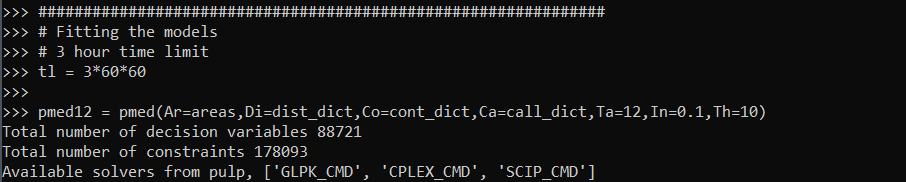Writing the class this way allows the user to input their own solver, and you can see it prints out the available solvers on your current machine. So to pass in the SCIOPT solver you could do `pmed12.solve(solver=pulp.SCIP_CMD())`, which again for this problem does find the solution, but takes 5 hours. So here I still stick with CPLEX to just illustrate the new classes functionality:

``pmed12.solve(solver=pulp.CPLEX(timeLimit=30*60,msg=True))     # takes around 10 minutes``

This prints out a ton of information at the command line that you do not get if you run from within Jupyter notebooks. So for debugging that is much more useful. `timeLimit` is in seconds, but does not include the presolve phase I believe, so some of these solvers can just get stuck on the presolve forever.

We can look at the results in a nice map if you do have geopandas installed and pass it a geopandas data frame and the key to match it on:

``pmed12.map_plot(carr_report, 'PDGrid')``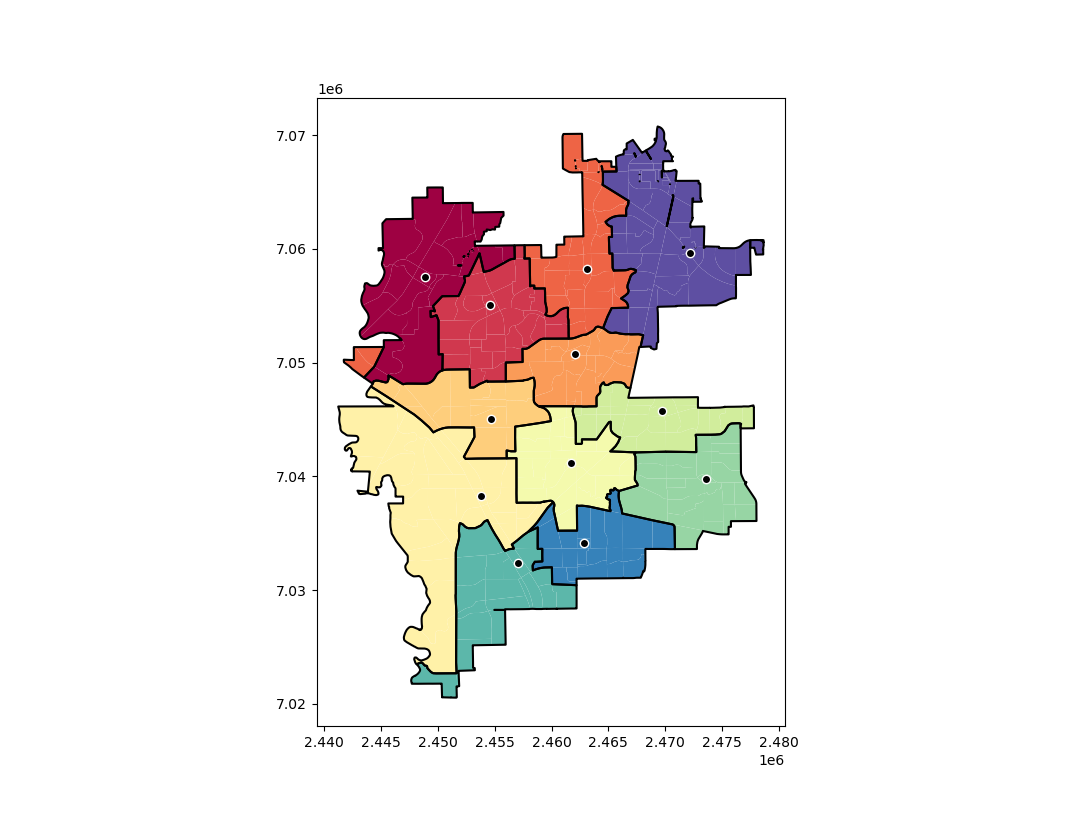This map shows the new areas and different colors with a thick border, and the source area as a dot with a white outline. So here you can see that we end up having one disconnected area – a subtour in optimization parlance. I have added some methods to deal with this though:

``stres = pmed12.collect_subtours()``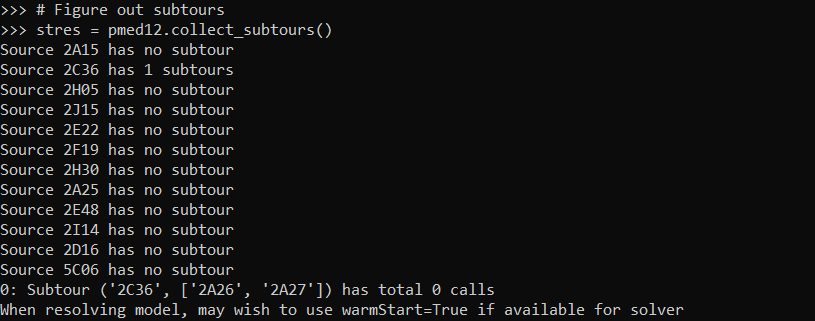And you can see that for one of our areas it identified a subtour. I haven’t written this to automatically resolve for subtours due to the potential length of the solving time. So here we can see the subtours have 0 calls in them. You can assign those areas to wherever and it does not change the objective function. So that is what I did in the paper and showed how in the Jupyter notebook at the main page for the github project.

So if you are using a function that takes 5 hours, I would suggest you manually fix these subtours if there is an obvious to the human eye easy solution. But here with the shorter solve time with CPLEX I can rerun the algorithm with a warm start, and it runs even faster (under 5 minutes). The `.collect_subtours()` method adds subtour constraints into the pulp model, so you just need to redo the `solve()` method to eliminate that particular subtour (I do not know if this is guaranteed to converge though for all problems).

So here in this data eliminating that one subtour results in a solution with no subtours:

``````pmed12.solve(solver=pulp.CPLEX_CMD(msg=False,warmStart=True))
stres = pmed12.collect_subtours()``````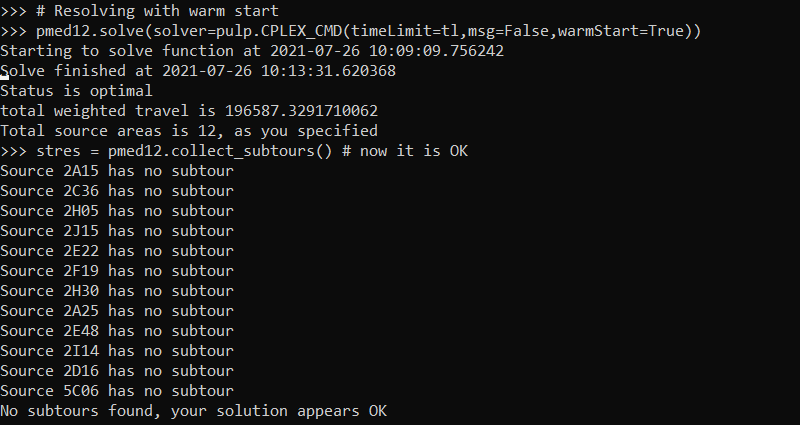And we can replot the result, which shows it chooses the areas that you would have assigned by eye anyway:

``pmed12.map_plot(carr_report, 'PDGrid')``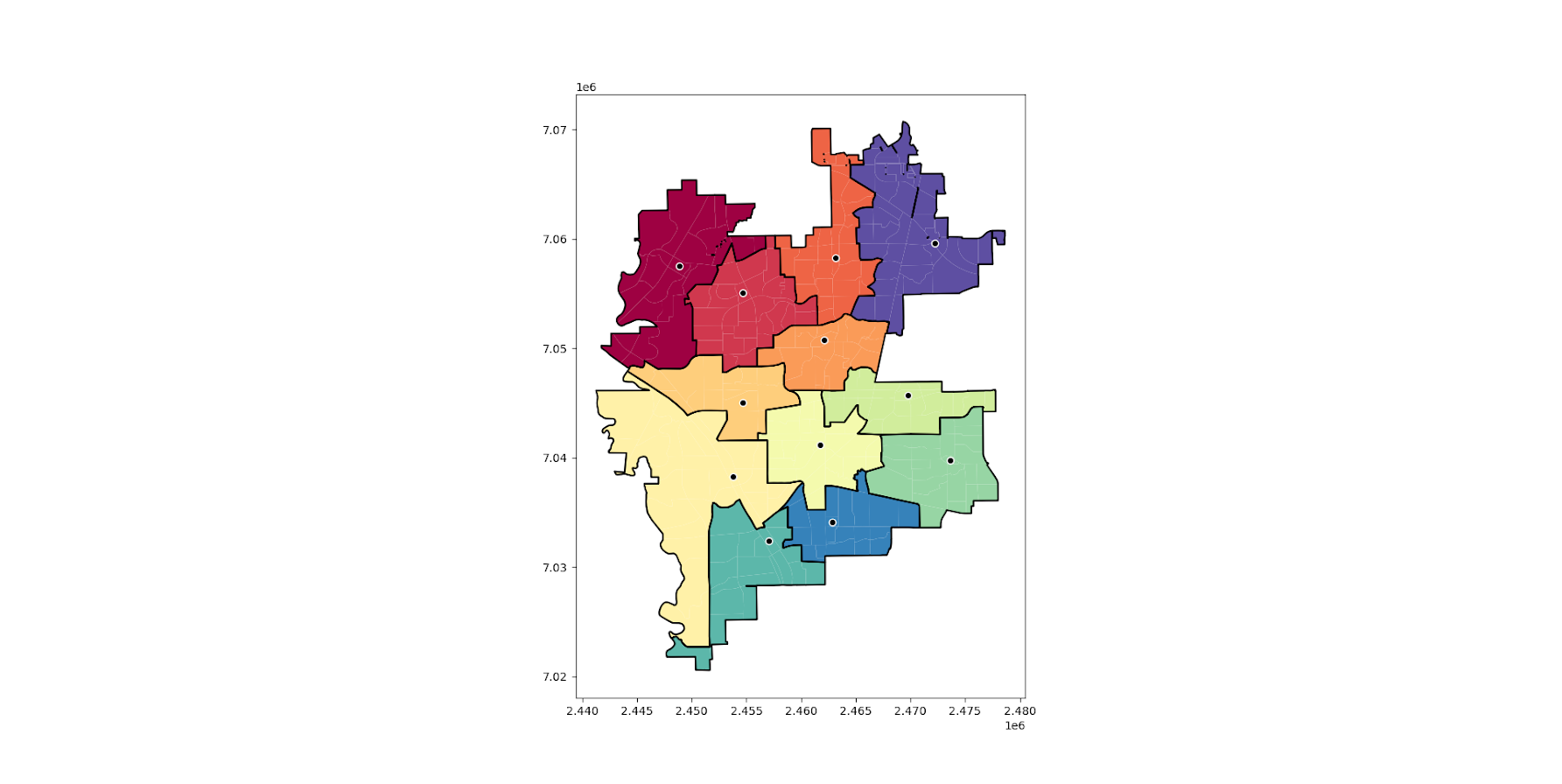So again for this problem if you have CPLEX I would recommend it (have not tried Gurobi). But at least for this particular dataset SCIOPT was able to solve the problem in 5 hours. So if you are a crime analyst or someone else without academic access to CPLEX you can install the sciopt solver and give that a go for your actual data.

Note that this is based off of results for 325 subareas. So if you have more subareas it will take a longer (and if you have fewer it may be quite a bit shorter).

# Setting up the Conda environment

So once you have python installed, you can typically do something like:

``pip install pulp``

Or via conda:

``conda install pyscipopt``

I often have trouble though, especially when working with the python geospatial libraries, to install geopandas, fiona, etc. So here what I do is create a new conda environment:

``````conda create -n linprog
conda activate linprog
conda install -c conda-forge python=3 pip pandas numpy networkx scikit-learn dbfread geopandas glpk pyscipopt pulp``````

And then you can run the pmedian code above in this environment. I suppose I should turn this into a python package, and I see a bunch of folks anymore are doing docker images as well with their packages in complicated environments. This is actually not that bad, minus geopandas makes things a bit tricky.

# Clumpy hotspots

Read an article by Tim Hart the other day (part of a special issue I will have an article in as well here soon). In it he evaluated hot spot methods not only by how well they forecast crime, but also by the clumpiness of the hot spot method. Some hot spot methods, such as risk terrain modeling (Caplan et al., 2020; Fox et al., 2021), machine learning models (Wheeler & Steenbeek, 2020), or self-exciting point process models (Mohler et al., 2018) can by their nature produce discontinuous hot spots. Here is an example of a RTM map I made in Yoo & Wheeler (2019) for homeless related crime in Los Angeles, and you can see this is quite spotty in the ups/downs in the high risk areas:Other hot spot methods, like hierarchical clustering (Wheeler & Reuter, 2020) or kernel density maps however this is not as big an issue. Here is an example kernel density map also from Yoo & Wheeler (2019) based on the same data:So you can see how the hot spots in the kernel density map are spatially contiguous, whereas the RTM example can be little hot spots all over the jurisdiction. So it is obviously easier to patrol a single contiguous area than many islands over the entire jurisdiction. So it may make sense to trade off a contiguous area that captures somewhat fewer crimes than speckled areas that are all over the map.

Adepeju et al. (2016) was the first to use a particular statistic, the clumpiness index, to evaluate different hot spot methods. Their figure below is a pretty good depiction of the idea – count up the number of internal edges to a hot spot (when a hot spot grid-cell neighbors another hot spot), and the number of external edges. Then it is just a particular formula to make the index range from -1 to 1 given different sized hot spots.So here I flip this idea on its head abit – instead of using a particular hot spot technique and see its clumpiness, I formulate a linear program given a prediction to trade off a smaller number of predicted crimes in the hot spot vs making the hot spot areas more clumpy. I illustrate my clumpy hot spots using just prior data to predict future data, in particular thefts from motor vehicles in Raleigh North Carolina.

I have posted the data/code on github here. It is a bit too long to embed the code directly in the blog post, but just see the `00_PrepData.py` file. The crime data and Raleigh border I downloaded from the Raleigh open data website.

# A Linear Program to Clump Hot Spots

So for some quick and dirty math in text, the linear program I formulate is:

``````Maximize { Sum[ theta*S_i*Crime_i + (1 - theta)*E_i ] }
Subject To:
1) Sum( S_i ) = k
2) E_i <= Sum(S_n for n in neighbors(i) ) for each i
3) E_i <= S_i for each i
4) S_i element of {0,1}, E_i >= 0 (and can be continuous)``````

The idea behind this is that if `theta=1`, this is the same as just taking whatever your input areas are and ranking them to pick the top `k` areas. So if you have 10000 500 by 500 foot grid cells as your spatial units of analysis, and you wanted the top 1% of the city, that is 100 grid cells. So you would choose `k=100` in that scenario. `Crime_i` here I use as prior counts of crime in the grid cell, but it could be the predicted value from whatever model as well. That is the first constraint in this model approach – you need to choose the total area you want. `S_i` are the decision variables for the final selected hot spot areas.

The second and third constraints determine the values for the second set of decision variables, `E_i`. These are the decision variables that encode the interconnected links when a selected grid cell touches another grid cell. Constraint 2 sets `E_i` to the total number of neighbors of `i` that are selected, except constraint 3 says if `S_i` is 0 `E_i` needs to be 0 as well.

In this formulation, `S_i` need to be integer variables, but the `E_i` are defined by the sum of `S_i`, so they can be continuous. In this formulation if you have N grid cells (or whatever spatial units of analysis), this results in `2*N` decision variables, and `2*N + 1` constraints. You could maybe save a few constraints here by working with an undirected graph instead of a directed one (in essence this double counts, a-b and b-a would count as two links). But this will just make it `1.5*N` constraints instead of `2*N`. So not a big deal probably. I did have some issues solving this using pulps default coin/GLPK solver. But CPLEX solved it no problem. (My example is a total of 20,986 500 by 500 foot grid cells, and I use rook contiguity like the Adepeju article as well. And using CPLEX it solves the models in just a few seconds.)

In this formulation you can think of `theta` as trading off crimes in the hot spot vs interior edges. So imagine you had `theta=0.9`, and you had a solution with 200 crimes and 100 interior edges. The objective function in that scenario would be `0.9*200 + 0.1*100 = 190`. Now imagine you had an alternative scenario with 190 crimes, but 200 internal edges, the objective function would be `0.9*190 + 0.1*200 = 191`. So you are saying, it is ok to have hot spots capture a smaller number of crimes, if they are more connected.

# Normal Hotspots vs Clumpy Ones in Raleigh

The open data I use for Raleigh, North Carolina for the NIBRS dataset goes back to June 2014, and has data updated in the beginning of March 2021. I pull out larcenies from motor vehicles, and for the historical train dataset use car larcenies from 2014 through 2019 (n = 17,681). For the test dataset I use car larcenies in 2020 and what is available so far in 2021 (n = 3,376). Again these are grid cells generated over the city boundaries at 500 by 500 foot intervals. For illustration I grab out the top 1% of the city (209 grid cells). I use a train/test dataset as out of sample test data will typically result in reduced predictions. Here are the PAI stats for train vs test when selecting the top 1%.For all subsequent selections I always use the historical training data to select the hot spots, and the test dataset to evaluate the PAI.

If we do the typical approach of just taking the highest crime grid cells based on the historical data, here are the results both for the PAI and the CI (clumpy index). For those not familiar, PAI is `% Crime Capture/% Area`, so if the denominator is 1%, and the PAI (for the test data) is 17, that means the hot spots capture 17% of the total thefts from vehicles. The CI ranges from -1 (spread apart) to 1 (entirely clustered). Here it is just over 0, suggesting these are basically randomly distributed in terms of clustering.You may think that almost spatial randomness in terms of clumping seems at odds with that crime clusters! But it is not really – a consistent relationship with crime hot spots is that they are intensely localized, and often you can go down the street and be in a low crime area (Harries, 2006). The same idea when people say high crime neighborhoods often are spotty interior – they tend to have mostly low crime areas and just a few specific hot spots.

OK, so now to show off my linear program. So what happens if we use `theta=0.9`?The total crime numbers are here for the historical data, and it ends up capturing the exact same number of crimes as the select top 1% does (3,664). But, it switches the selection of one of the areas. So what happens here is that we have ties – even with basically little weight assigned to the interior connections, it will prioritize tied crime areas to be connected to other chosen hot spots (whereas before the ties are just random in the way I chose the top 1%). So if you have many ties at the threshold for your hot spot, this is a great way to prioritize particular tied areas.

What happens if we turn down theta to 0.5? So this is saying you would trade off one for one – one interior edge is equal to one crime.You can see that it changed the selections slightly more here, traded off 24 areas compared to the original just rank solution. Lets check out the map and the CI:The CI value is now 0.17 (up from 0.08). You can see some larger blobs, but it is still pretty spread apart. But the reduction in the total number of crimes captured is pretty small, going from a PAI of 17 to now a PAI of 16. How about if we crank down theta even more to 0.2?This trades off a much larger number of areas and total amount of crime – over half of the chosen grid cells are flipped in this scenario. In the subsequent map you can see the hot spots are much more clumpy now, and have a CI of 0.64.The PAI of 12.6 is a bit of a hit as well, but is not too shabby still. I typically take a PAI of 10 to be the ballpark of what is reasonable based on Weisburd’s Law of Crime Concentration – 5% of the areas contain 50% of the crime (which is a PAI of 10).

So this shows one linear programming approach to trade off clumpy chosen areas vs disconnected speckles over the map. It may be the case though that other approaches are more reasonable, such as using some type of clustering to begin with. E.g. I could use DBSCAN on the gridded predicted values (Wheeler & Reuter, 2020) as see how clumpy those hot spots are. This approach is nice though if you have a fixed area you want to cover though.

# Why Raleigh?

For a bit of personal news, I will be moving to the Raleigh area here shortly. I recently negotiated to be 100% remote at my job – so I will still be at HMS (or since we were recently purchased I might be employed by Gainwell I guess by the time I move). So looking forward to the new adventure back on the east coast but still in more temperate climates than PA or NY!

# Incorporating treatment non-compliance into call-ins

I have previously published work on identifying optimal individuals to prioritize for call-ins in Focused Deterrence interventions. The idea is we want to identify optimal people to spread the message, so you call in a small number of individuals and they should spread the message to the remaining group. There are better people than others to seed the message to to make sure it spreads throughout the network.

I knew of a direct improvement on that algorithm I published (very similar to the TURF problem I described the other day). But the bigger issue was that even when you call in individuals they do not always come to the meeting – treatment non-compliance. When working with state parole and/or local probation, the police department can ask those agencies to essentially make people come in, but otherwise it is voluntary.

The TURF problem I did the other day gave me a bit of inspiration on how to tackle that treatment non-compliance problem though. In a nutshell when you calculate whether someone is reached (via being directly connected to someone called-in), they can be partially reached based on the probability of the selected nodes treatment compliance. I have posted the code to follow along on dropbox here. I won’t go through the whole thing, but just some highlights.

# The Model

First, in some quick and dirty text math, the model is:

`Maximize Sum( R_i )`

Subject to:

• `R_i <= Sum( S_j*p_j ) for each i`
• `Sum( S_j ) = k`
• `S_i element of [0,1]`
• `R_i <= 1 for each i`

Here `i` refers to an individual node in the gang/group network.

The first constraint `R_i <= Sum( S_j*p_j )`, the j’s are the nodes that are connected to i (and i itself). The p_j are the estimates that an individual will comply with coming into the call-in. For one agency we worked with for that project, they guessed that those who don’t need to come in comply about 1/6th of the time, so I use that estimate here in my examples, and give people who are on probation/parole a 1 for the probability of compliance.

Second constraint is we can only call in so many people, here `k`. The model solves very fast, so you can generate results for various k until you get the reach you want to in the end. (You could do the model the other way, minimize `S_i` while constraining the minimized acceptable reach, e.g. `Sum( R_i ) >= threshold`, I don’t suggest this in practice though, as when dealing with compliance there may be no feasible solution that gets you the amount of reach in the network you want.)

For the third constraint, the decision variables `S_i` are binary 0/1’s, but the `R_i` are continuous. But the trick here is that the last constraint, `R_i <= 1`, means that the expected reach is capped at 1. Here is a way to think about this, imagine you want to know the chance that person A is reached, and they are connected to two called-in individuals, who each have a 40% chance at complying with the treatment (coming to the call-in). The expected times person A would be reached then is additive in the probabilities, `0.4 + 0.4 = 0.8`. If we had 3 people connected to A again at 40% apiece, the expected number of times A would be reached is then `0.4 + 0.4 + 0.4 = 1.2`. So a person can be reached multiple times. (Note this is not the probability a person is reached at least once! It is a non-linear problem to model that.)

But if we took away the last constraint, what would happen is that the algorithm would just pick the nodes that had the highest number of neighbors. Since we are maximizing expected reach, if we had a sample of two people, the expected reach values of `[2.5, 0]` would be preferable to `[1, 1]`, although clearly we rather have the reach spread out. So to prevent that, I cap the expected reach variable at 1, `R_i <= 1 for each i`, so this spreads out the selected individuals. So in the end the expected number of times people are reached are a lower bound estimate, but those are only people who are expected to receive the message multiple times.

This is a bit of a hack, but in my tests works quite well. I attempted to model the non-linear problem of estimating the probabilities at the person level and still maximizing the expected reach (in the code I have an example of using the `CVXR` R package). But it was quite fickle in when it would return a solution. So I am focusing on the linear program here, which is not perfect, but is an improvement over my prior published work.

# Some Python Snippets

So for my example code, I am using City 4 Gang 4 from my paper. The reason is this was the largest network, and my original algorithm performed the worst. 99 nodes, and my original algorithm identified a 33 person dominant set, but Borgotti’s tool (that uses a genetic algorithm) identified a 29 dominant set.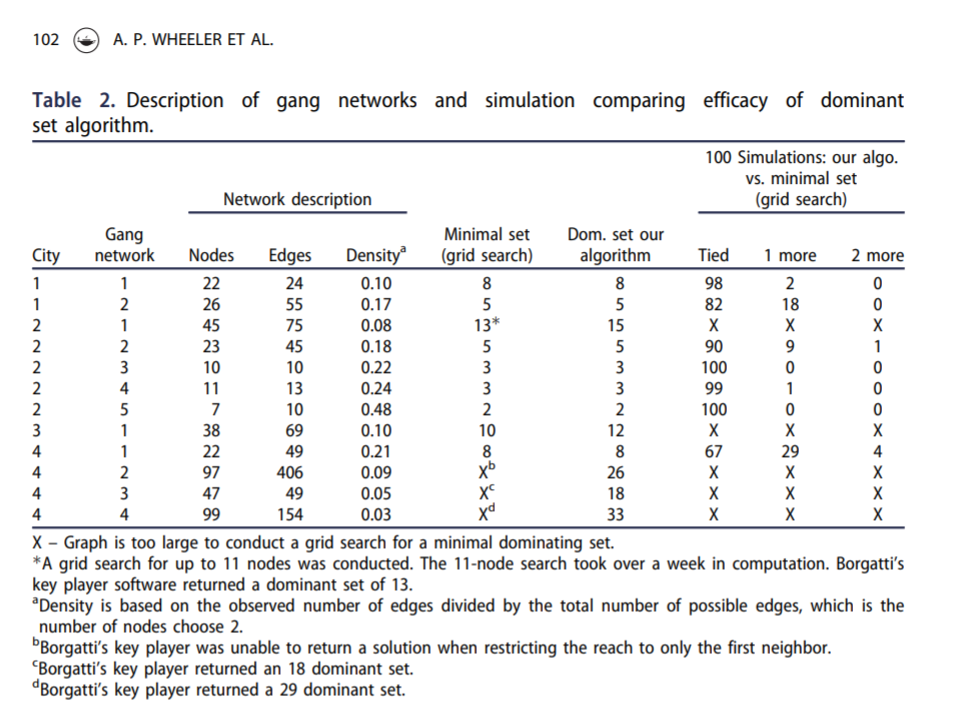Here is an example of calling my function to select the individuals for a call-in based on the non-compliance estimates. (`g4` is the networkx graph object, the second arg is the number of individuals, and `compliance` is the node attribute that has the probability of treated compliance.) If we call in only 5 people, we still expect a reach of 29 individuals. Here there ends up being some highly connected people on parole/probation, so they have a 1 probability of complying with the treatment.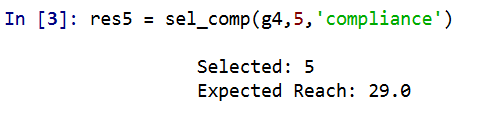A consequence of this algorithm is that if you pipe in 1’s for the treatment compliance, you basically get an improvement to my original algorithm. So for a test we can see if I get the same minimal dominating set as Borgotti did for his algorithm here, where `const` is just everybody complies 100% of the time.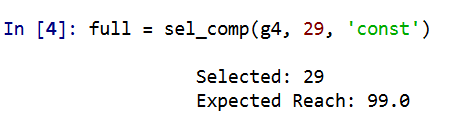And yep we get a dominating set (all 99 people are reached). What happens if we go down one, and only select 28 people?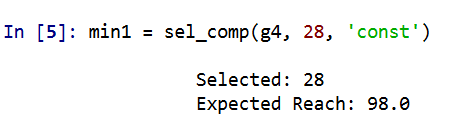We only reach 98 out of the 99. So it appears a 29 set is the minimal dominating set here. But like I said the treatment non-compliance is a big deal in this setting. What is our expected reach if we take that into account, but still call-in 29 people?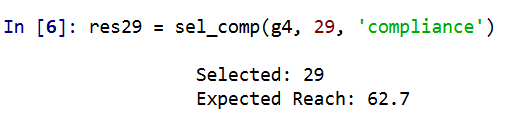It is still pretty high, around 2/3s of the network, but is still much smaller. Also if you look at the overlap between the constant versus non-compliance model, they select quite a few different individuals. It makes a big difference.

Here is a graph I made of selecting 20 individuals. Red means I selected that person, pink means they are reached at least some, and the size of the reach is proportion to the node. Then grey folks I wouldn’t expect to be reached by the message (at least by first degree connections).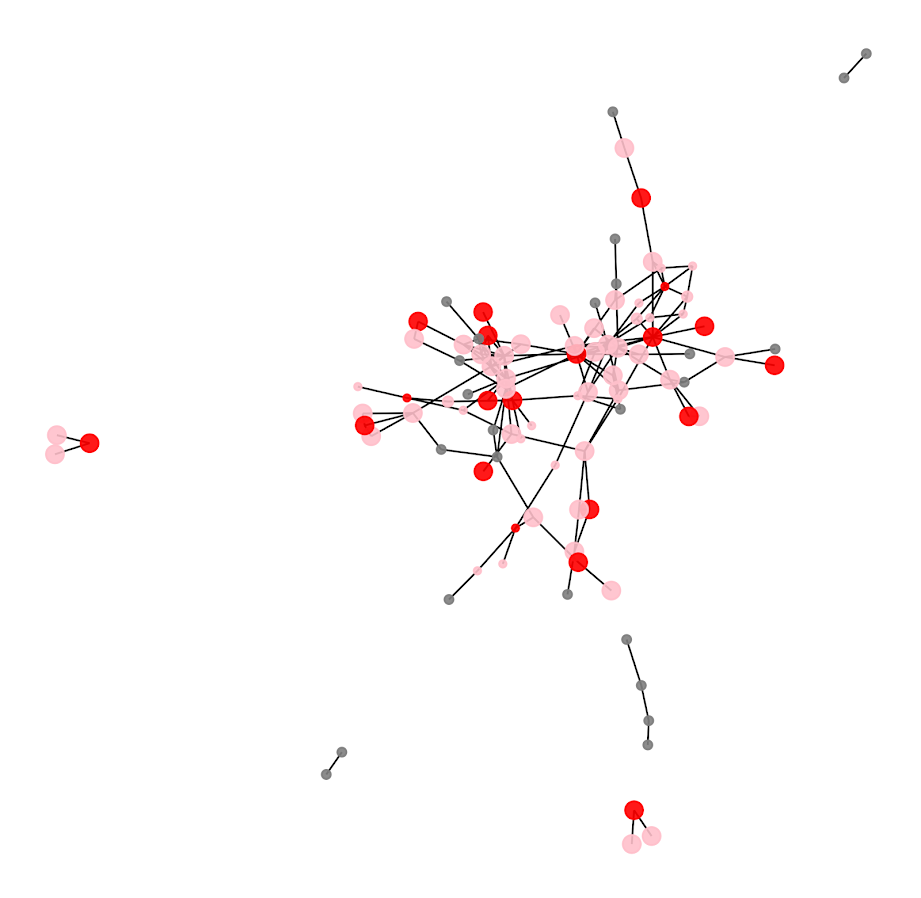So you can see that most of the people selected have that full 1 expected reach, so the algorithm does prioritize individuals on probation/parole who have a 100% expected compliance. But you can see a few folks who have a lower compliance who are selected as they are in places in the network not covered by those on probation/parole.

I have a tough time getting network layouts to look nice in python (even with the same layout algorithms, I feel like igraph in R just looks much better out of the box).

# Future Work

Out of the box, this algorithm could incorporate several different pieces of information. So here I use the non-compliance estimate as a constant, but you could have varying estimates for that based on some other model no problem (e.g. older individuals comply more often than younger, etc.). Also another interesting extension (if you could get estimates) would be the probability a called-in individual spreads the message. In the part `Sum( S_j*p_j )` it would just be something like `Sum( S_j*p_cj*p_sj )`, where p_cj is the compliance probability for attending, and p_sj is the probability to spread the message to those they are connected to.

Getting worthwhile estimates for either of those things will be tough though. Only way I can see it is via some shoe leather qualitative or survey approach.

# A linear programming example for TURF analysis in python

Recently on LinkedIn I saw a very nice example of TURF (Total Unduplicated Reach & Frequency) analysis via Jarlath Quinn. I suggest folks go and watch the video, but for a simple example imagine you are an ice cream food truck, and you only have room in your truck to sell 5 different ice-creams at a time. Some people only like chocolate, others like vanilla and neapolitan, and then others like me like everything. So what are the 5 best flavors to choose to maximize the number of people that like at least one flavor?

You may think this is a bit far-fetched to my usual posts related to criminal justice, but it is very much related to the work I did on identifying optimal gang members to deliver the message in a Focused Deterrence initiative. (I’m wondering if also there is an application in Association Rules/Conjunctive analysis.) But most examples I see of this are in the marketing space, e.g. whether to open a new store, or to carry a new product in a store, or to spend money on ads to reach an audience, etc.

Here I have posted the python code and data used in the analysis, below I go through the steps in formulating different linear programs to tackle this problem. I ended up taking some example simulated data from the XLStat website. (If you have a real data example feel free to share!)

A bit of a side story – growing up in rural Pennsylvania, going out to restaurants was sort of a big event. I specifically remember when we would travel to Williamsport, we would often go to eat at a restaurant called Hoss’s and we would all just order the salad bar buffet. So I am going to pretend this restaurant survey is maximizing the reach for Hoss’s buffet options.

# Frontmatter

Here I am using pulp to fit the linear programming, reading in the data, and I am making up names for the columns for different food items. I have a set of main course meals, sides, and desserts. You will see in a bit how I incorporate this info into the buffet plans.

``````############################################################
#FRONT MATTER

import pandas as pd
import pulp
import os

os.chdir('D:\Dropbox\Dropbox\Documents\BLOG\TURF_Analysis\Analysis')

#This is simulated data from XLStat

#Need 27 total of match up simulated data
main = ['Steak',
'Pizza',
'FriedChicken',
'BBQChicken',
'GrilledSalmon',
'Roast',
'Burger',
'Wings']

'TomatoSoup',
'OnionSoup',
'Peas',
'BrusselSprouts',
'GreenBeans',
'Corn',
'DeviledEggs',
'Pickles']

desserts = ['ChoclateIceCream',
'VanillaIceCream',
'Brownie',
'Blondie',
'CherryPie']

#Renaming columns
surv_data.columns = main + sides + desserts

#Replacing the likert scale data with 0/1
surv_data.replace([1,2,3],0,inplace=True)
surv_data.replace([4,5],1,inplace=True)

#A customer weight example, here setting to 1 for all
surv_data['CustWeight'] = 1
cust_weight = surv_data['CustWeight']
############################################################``````

# Maximizing Customers Reached

And now onto the good stuff, here is an example TURF model linear program. I end up picking the same 5 items that the XLStat program picked in their spreadsheet as well.

``````############################################################

k = 5 #pick 5 items
Cust_Index = surv_data.index
Prod_Index = main + sides + desserts

#Problem and Decision variables
P = pulp.LpProblem("TURF", pulp.LpMaximize)
Cust_Dec = pulp.LpVariable.dicts("Customers Reached", [i for i in Cust_Index], lowBound=0, upBound=1, cat=pulp.LpInteger)
Prod_Dec = pulp.LpVariable.dicts("Products Selected", [j for j in Prod_Index], lowBound=0, upBound=1, cat=pulp.LpInteger)

#Objective Function
P += pulp.lpSum( Cust_Dec[i] * cust_weight[i] for i in Cust_Index )

surv_items = surv_data[Prod_Index] #Dont want the weight variable
#Reached Constraint
for i in Cust_Index:
#Get the products selected
p_sel = surv_items.loc[i] == 1
sel_prod = list(p_sel.index[p_sel])
#Set the constraint
P += Cust_Dec[i] <= pulp.lpSum( Prod_Dec[j] for j in sel_prod )

#Total number of products selected constraint
P += pulp.lpSum( Prod_Dec[j] for j in Prod_Index) == k

#Now solve the model
P.solve()

#Figure out the total reached people
print( pulp.value(P.objective) ) #129

#Print out the products you picked
picked = []
for n,j in enumerate(Prod_Index):
if Prod_Dec[j].varValue == 1:
picked.append( (n+1,j) )

print(picked)

#Same as XLStat
#[(14, 'OnionSoup'), (15, 'Peas'), (16, 'BrusselSprouts'),

#For 5 items, XLStat selected items
# 14 15 16 23 26 that reached 129 people
############################################################``````

One of the things I have done here is to create a ‘weight’ variable associated with each customer. So here I say all of the customers weights are all equal to 1, but you could swap out whatever you wanted. Say you had estimates on how much different individuals spend, so you could give big spenders more weight. (In a criminal justice example, for the Focused Deterrence initiative, folks typically want to target ‘leaders’ more frequently, so you may give them more weight in this example.) Since these examples are based on surveys, you may also want the weight to correspond to the proportion that survey respondent represents in the population, aka raking weights. Or if you have a crazy large survey population, you could use frequency weights for responses that give the exact same picks.

One thing to note as well in this formula is that I recoded the data earlier to be 0/1. You might however consider the likert scale rating 1 to 5 directly, subtract 1 and divide by 4. Then take that weight, and instead of the line:

``Cust_Dec[i] <= pulp.lpSum( Prod_Dec[j] for j in sel_prod )``

You may want something like:

``Cust_Dec[i] <= pulp.lpSum( Prod_Dec[j]*likert_weight[i,j] for j in sel_prod )``

In that case you would want to set the `Cust_i` decision variable to a continuous value, and then maybe cap it at 1 (so you can partially reach customers).

The total number of decision variables will be the number of customers plus the number of potential products, so here only `185 + 27 = 212`. And the number of constraints will be the number of customers plus an additional small number. I’d note you can easily solve systems with 100,000’s of decision variables and constraints on your laptop, so at least for the example TURF analyses I have seen they are definitely within the ‘can solve this in a second on a laptop’ territory.

You can add in additional constraints into this problem. So imagine we always wanted to select one main course, at least two side dishes, and no more than three desserts. Also say you never wanted to pair two items together, say you had two chicken dishes and never wanted both at the same time. Here is how you could do each of those different constraints in the problem.

``````############################################################
#CONSTRAINTS ON ITEMS SELECTED

#Redoing the initial problem, but select 7 items
k = 7
P2 = pulp.LpProblem("TURF", pulp.LpMaximize)
Cust_Dec2 = pulp.LpVariable.dicts("Customers Reached", [i for i in Cust_Index], lowBound=0, upBound=1, cat=pulp.LpInteger)
Prod_Dec2 = pulp.LpVariable.dicts("Products Selected", [j for j in Prod_Index], lowBound=0, upBound=1, cat=pulp.LpInteger)
P2 += pulp.lpSum( Cust_Dec2[i] * cust_weight[i] for i in Cust_Index )
for i in Cust_Index:
p_sel = surv_items.loc[i] == 1
sel_prod = list(p_sel.index[p_sel])
P2 += Cust_Dec2[i] <= pulp.lpSum( Prod_Dec2[j] for j in sel_prod )
P2 += pulp.lpSum( Prod_Dec2[j] for j in Prod_Index) == k

#No Fried and BBQ Chicken at the same time
P2 += pulp.lpSum( Prod_Dec2['FriedChicken'] + Prod_Dec2['BBQChicken']) <= 1
#Exactly one main course
P2 += pulp.lpSum( Prod_Dec2[m] for m in main) == 1
#At least two sides (but could have 0)
P2 += pulp.lpSum( Prod_Dec2[s] for s in sides) >= 2
#No more than 3 desserts
P2 += pulp.lpSum( Prod_Dec2[d] for d in desserts) <= 3

#Now solve the model and print results
P2.solve()
print( pulp.value(P2.objective) ) #137
picked2 = []
for n,j in enumerate(Prod_Index):
if Prod_Dec2[j].varValue == 1:
picked2.append( (n+1,j) )
print(picked2)
#[(10, 'Wings'), (12, 'IcebergSalad'), (14, 'OnionSoup'), (15, 'Peas'),
# (16, 'BrusselSprouts'), (23, 'ChocChipCookie'), (27, 'CherryPie')]
############################################################``````

You could also draw a trade-off curve for how many more people you will reach if you can up the total number of items you can place on the menu, so estimate the model with 4, 5, 6, etc items and see how many more people you can reach if you extend the menu.

One of the other constraints you may consider in this formula is a budget constraint. So imagine instead of the food example, you are working for a marketing company, and you have an advertisement budget. You want to maximize the customer reach given the budget, so here a “product” may be a billboard, radio ad, newspaper ad, etc, but each have different costs. So instead of the constraint `Prod_j == k` where you select so many products, you have the constraint `Prod_j*Cost_j <= Budget`, where each product is associated with a particular cost.

# Alt Formula, Minimizing Cost while Reaching a Set Amount

So in that last bit I mentioned costs for selecting a particular portfolio of products. Another way you may think about the problem is minimizing cost while meeting constraints on the reach (instead of maximizing reach while minimizing cost). So say you were a marketer, and wanted an estimate of how much budget you would need to reach a million people. Or going with our buffet example, imagine we wanted to appeal to at least 50% of our sample (so at least 93 people). Our formula would then be below (where I make up slightly different costs for buffet each of the buffet options).

``````############################################################
#MINIMIZE COST

cost_prod = {'Steak' : 5.0,
'Pizza' : 2.0,
'FriedChicken' : 4.0,
'BBQChicken' : 3.5,
'GrilledSalmon' : 4.5,
'Roast' : 3.9,
'Burger' : 1.5,
'Wings' : 2.4,
'TomatoSoup' : 0.4,
'OnionSoup' : 0.9,
'Peas' : 0.6,
'BrusselSprouts' : 0.5,
'GreenBeans' : 0.4,
'Corn' : 0.3,
'DeviledEggs' : 0.7,
'Pickles' : 0.73,
'ChoclateIceCream' : 1.3,
'VanillaIceCream' : 1.2,
'Brownie' : 1.2,
'Blondie' : 1.3,
'CherryPie' : 1.9}

#Setting up the model with the same selection constraints
n = 100
Pmin = pulp.LpProblem("TURF", pulp.LpMinimize)
Cust_Dec3 = pulp.LpVariable.dicts("Customers Reached", [i for i in Cust_Index], lowBound=0, upBound=1, cat=pulp.LpInteger)
Prod_Dec3 = pulp.LpVariable.dicts("Products Selected", [j for j in Prod_Index], lowBound=0, upBound=1, cat=pulp.LpInteger)
#Minimize this instead of Maximize reach
Pmin += pulp.lpSum( Prod_Dec3[j] * cost_prod[j] for j in Prod_Index )
for i in Cust_Index:
p_sel = surv_items.loc[i] == 1
sel_prod = list(p_sel.index[p_sel])
Pmin += Cust_Dec3[i] <= pulp.lpSum( Prod_Dec3[j] for j in sel_prod )
#Instead of select k items, we want to reach at least n people
Pmin += pulp.lpSum( Cust_Dec3[i]*cust_weight[i] for i in Cust_Index) >= n

#Same constraints on meal choices
Pmin += pulp.lpSum( Prod_Dec3['FriedChicken'] + Prod_Dec3['BBQChicken']) <= 1
Pmin += pulp.lpSum( Prod_Dec3[m] for m in main) == 1
Pmin += pulp.lpSum( Prod_Dec3[s] for s in sides) >= 2
Pmin += pulp.lpSum( Prod_Dec3[d] for d in desserts) <= 3

#Now solve the model and print results
Pmin.solve()

reached = 0
for i in Cust_Index:
reached += Cust_Dec3[i].varValue
print(reached) #100 reached on the nose

picked = []
for n,j in enumerate(Prod_Index):
if Prod_Dec3[j].varValue == 1:
picked.append( (n+1,j,cost_prod[j]) )
cost += cost_prod[j]
print(picked)
#[(9, 'Burger', 1.5), (13, 'TomatoSoup', 0.4), (15, 'Peas', 0.6)]
print(pulp.value(Pmin.objective)) #Total Cost 2.5
############################################################``````

So for this example our minimum budget buffet has some burgers, tomato soup, and peas. (Sounds good to me, I am not a picky eater!)

You can still incorporate all of the same other constraints I discussed before in this formulation. So here we need at a minimum to serve only 3 items to get the (over) 50% reach that we desire. If you wanted fairness type constraints, e.g. you want to reach 60% of females and 40% of males, you could do that as well. In that case you would just have two separate constraints for each group level you wanted to reach (which would also be applicable to the prior maximize reach formula, although you may need to keep upping the number of products selected before you identify a feasible solution).

In the end you could mash up these two formulas into one bi-objective function. You would need to define a term though to balance reach and cost. I’m wondering as well if there is a way to incorporate marginal benefits of sales into this as well, e.g. if you sell a Steak you may make a larger profit than if you sell a Pizza. But I am not 100% sure how to do that in this set up (even though I like all ice-cream, I won’t necessarily buy every flavor if I visit the shop). Similar for marketing adverts some forms may have better reach, but may have worse conversion rates.

# Creating high crime sub-tours

I was nerdsniped a bit by this paper, Targeting Knife-Enabled Homicides For Preventive Policing: A Stratified Resource Allocation Model by Vincent Hariman and Larry Sherman (HS from here on).

It in, HS attempt to define a touring schedule based on knife crime risk at the lower super output area in London. So here are the identified high risk areas: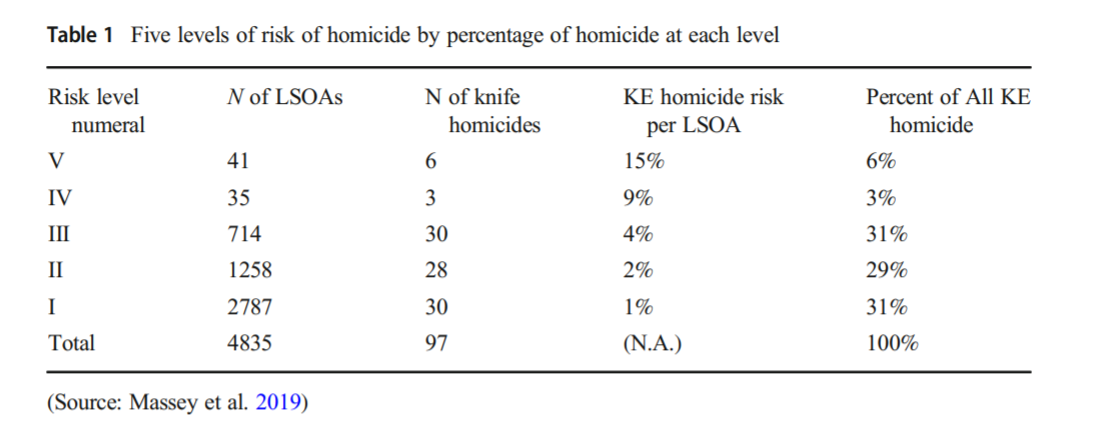And here are HS’s suggested hot spot tours schedule.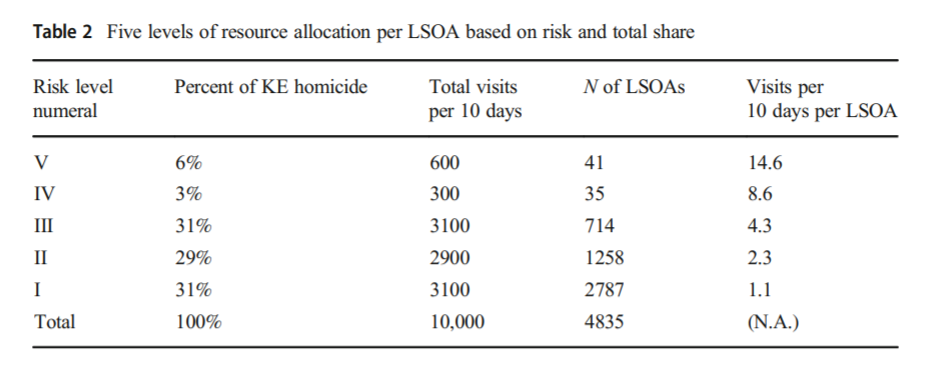This is ad-hoc, but an admirable attempt to figure out a reasonable schedule. As you can see in their tables, the ‘high’ knife crime risk areas still only have a handful of homicides, so if reducing homicides is the objective, this program is a bit dead in the water (I’ve written about the lack of predictive ability of the model here).

I don’t think defining tours to visit everywhere makes sense, but I do think a somewhat smaller in scope question, how to figure out geographically informed tours for hot spot areas does. So instead of the single grid cell target ala PredPol, pick out multiple areas to visit for hot spots. (I don’t imagine the 41 LSOA areas are geographically contiguous either, e.g. it would make more sense to pick a tour for areas connected than for areas very far apart.)

Officers don’t tend to like single tiny areas either really, and I think it makes more sense to widen the scope a bit. So here is my attempt to figure those reasonable tours out.

# Defining the Problem

The way I think about that problem is like this, look at the hypothetical diagram below. We have two choices for the hot spot location we are targeting, where the crime counts for locations are noted in the text label.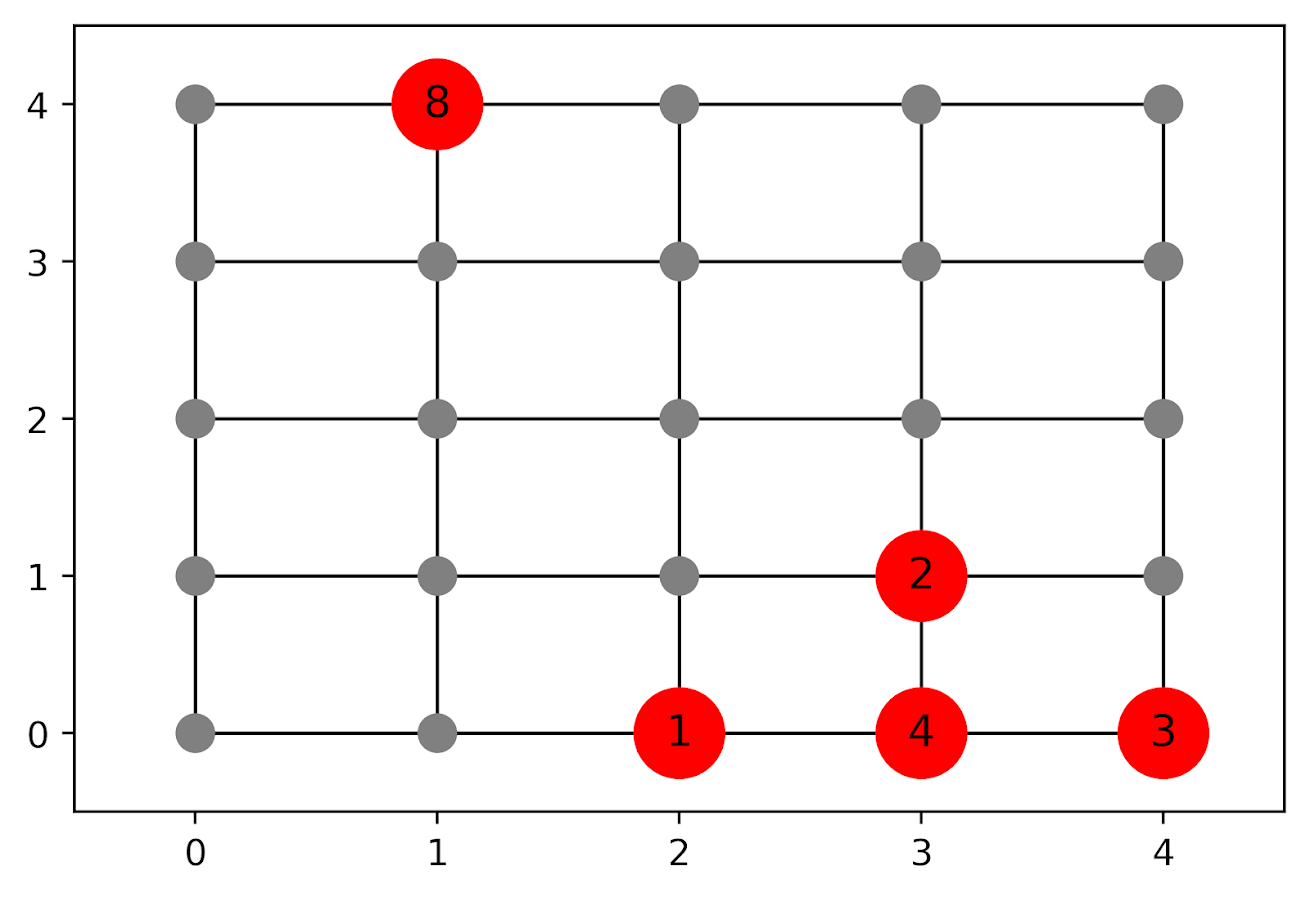In the select the top hot spot (e.g. PredPol) approach, you would select the singlet grid cell in the top left, it is the highest intensity. We have another choice though, the more spread out hot spot in the lower right. Even though it is a lower density, it ends up capturing more crime overall.

I subsequently formulated an integer linear program to try to tackle the problem of finding good sub-tours through the graph that cumulatively capture more crime. So with the above graph, if I select two subtours, I get the results as (where nodes are identified by their `(x,y)` position):

• `['Begin', (1, 4), 'End']`
• `['Begin', (4, 0), (4, 1), (3, 1), (3, 0), (2, 0), 'End']`

So it can select singlet areas if they are islands (the `(1,4)` area in the top left), but will grow to wind through areas. Also note that the way I have programmed this network, it doesn’t skip the zero area `(4,1)` (it needs to go through at least one in the bottom right unless it doubles back on itself).

I will explain the meaning of the begin and end nodes below in my description of the linear program. It ends up being sort of a mash-up of traveling salesman type vehicle routing and min cost max flow type problems.

# The Linear Program

The way I think about this problem formulation is like this: we have a directed graph, in which you can say, OK I start from location A, then can go to B, than go to C. In my set of decision variables, I have choices that look like this, where the first subscript denotes the from node, and the second subscript denotes the to node.

``````D_ab := node a -> node b
D_bc := node b -> node c``````

etc. In our subsequent linear program, the destination node is the node that we calculate our cumulative crime density statistics. So if node B had 10 crimes and 0.1 square kilometers, we would have a density of 100 crimes per square kilometer.

Now to make this formulation work, we need to add in a set of special nodes into our usual location network. These nodes I call ‘Begin’ and ‘End’ nodes (you may also call them source/sink nodes though). The begin nodes all look like this:

``````D_{begin},a
D_{begin},b
D_{begin},c``````

So you do that for every node in your network. Then you have End nodes as well, e.g.

``````D_a,{end}
D_b,{end}
D_c,{end}``````

In this formulation, since we are only concerned about the crime stats for the to node, not the from node, the Begin nodes just inherit the crime density stats from the original node data. For the end nodes though, you just set their objective value stats to zero (they are only relevant to define the constraints).

Now here is my linear program formulation:

``````Maximize
Sum [ D_ij ( CrimeDensity_j - DensityPenalty_j ) ]

Subject To:

1. Sum( D_in for each neighbor of n ) <= 1,
for each original node n
2. Sum( D_in for each neighbor of n ) =  Sum( D_ni for each neighbor of n ),
for each original node n
3. Sum( D_bi for each begin node ) = k routes
4. Sum( D_ie for each end node ) = k routes
5. Sum( D_ij + D_ji ) <= 1, for each unique i,j pair
6. D_ij is an element of {0,1}``````

Constraint 1 is a flow constraint. If a node has an incoming edge set to one, it cannot have any other incoming edge set to one (so a location can only be chosen once).

Constraint 2 is a constraint that says if an incoming node is selected, one of the outgoing edges needs to be selected.

Constraints 3 & 4 determine the number of k tours/routes to choose in the end. Since the begin/end nodes are special we have k routes going out of the begin nodes, and k routes going into the end nodes.

With just these constraints, you can still get micro-cycles I found. So something like, X -> Z -> X. Constraint 5 (for only the undirected edges) prevents this from happening.

Constraint 6 is just setting the decision variables to binary 0/1. So it is a mixed integer linear program.

The final thing to note is the objective function, I have `CrimeDensity_j - DensityPenalty_j`, so what exactly is DensityPenalty? This is a value that penalizes visiting areas that are below this threshold. Basically the way that this works is that, the density penalty sets an approximate threshold for the minimum density a tour should contain.

I suggest a default of a predictive accuracy index of 10. Where do I get 10 you ask? Weisburd’s law of crime concentration suggests 5% of the areas should contain 50% of the crime, which is a PAI of 0.5/0.05 = 10. In my example with DC data then I just calculate the actual density of crime per unit area that corresponds to a PAI of 10.

You can adjust this though, if you prefer smaller tours of higher crime density you would up the value. If you prefer longer tours decrease it.

This is the best way I could figure out how to trade off the idea of spreading out the targeted hot spot vs selecting the best areas. If you spread out you will ultimately have a lower density. This turns it into a soft objective penalty to try to keep the selected tours at a particular density threshold (and will scoop up better tours if they are available). For awhile I tried to figure out if I could maximize the PAI metric, but it is the case with larger areas the PAI will always go down, so you need to define the objective some other way.

This formulation I only consider linked nodes (unlike the usual traveling salesman in which it is a completely linked distance graph). That makes it much more manageable. In this formulation, if you have `N` as the number of nodes/areas, and `E` is the number of directed edges between those areas, we will then have:

• `2*N + E` decision variables
• `2 + 2*N + E/2` constraints

Generally if you are doing directly connected areas in geographic networks (i.e. contiguity connections), you will have less than 8 (typically more like an average of 6) neighbors per each area. So in the case of the 4k London lower super output areas, if I chose tours I would guess it would end up being fewer than `2*4,000 + 8*4,000 = 40,000` decision variables, and then fewer than that constraints.

Since that is puny (and I would suggest doing this at a smaller geographic resolution) I tested it out on a harder network. I used the data from my dissertation, a network of 21,506 street units (both street segments and intersections) in Washington, D.C. The contiguity I use for these micro units is based on the Voronoi tessellation, so tends to have more neighbors than you would with a strictly road based network connectivity. Still in the end it ends up being a shade fewer than 200k decision variables and 110k constraints. So is a better test for in the wild whether the problem can be feasibly solved I think.

# Example with DC Data

Here I have posted the python code and data used for this analysis, I end up having a nice function that you just submit your network with the appropriate attributes and out pops the different tours.

So I end up doing examples of 4 and 8 subtours based on 2011 violent UCR crime data (agg assaults, robberies, and homicides, no rapes in the public data). I use for the penalty that PAI = 10 threshold, so it should limit tours to approximately that value. It only ends up taking 2 minutes for the model to converge for the 4 tours and less than 2.5 minutes for the 8 tours on my desktop. So it should be not a big problem to up the decision variables to more sub-areas and still be solvable in real life applications.

The area estimates are in square meters, hence the high numbers. But on the right you can see that each sub-tour has a PAI above 10.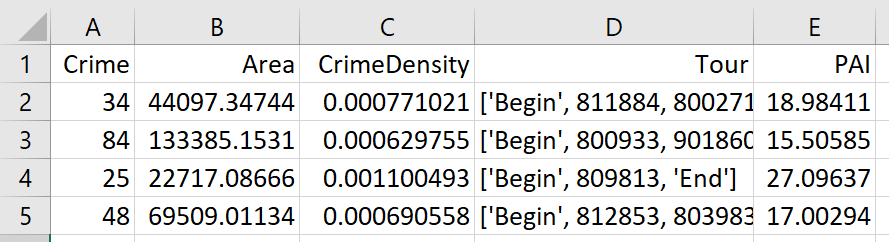Here is an interactive map for you to zoom into each 4 subtour example. Below is a screenshot of one of the subtours. You can see that since I have defined my connected areas in terms of Voronoi tessalations, they don’t exactly follow the street network.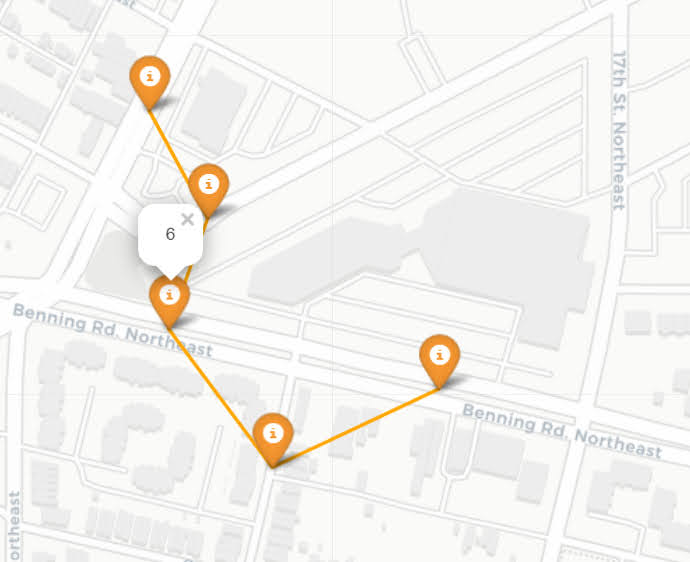For the 8 tour example, it ends up returning several zero tours, so it is not possible in this data to generate 8 sub-tours that meet that PAI >= 10 threshold.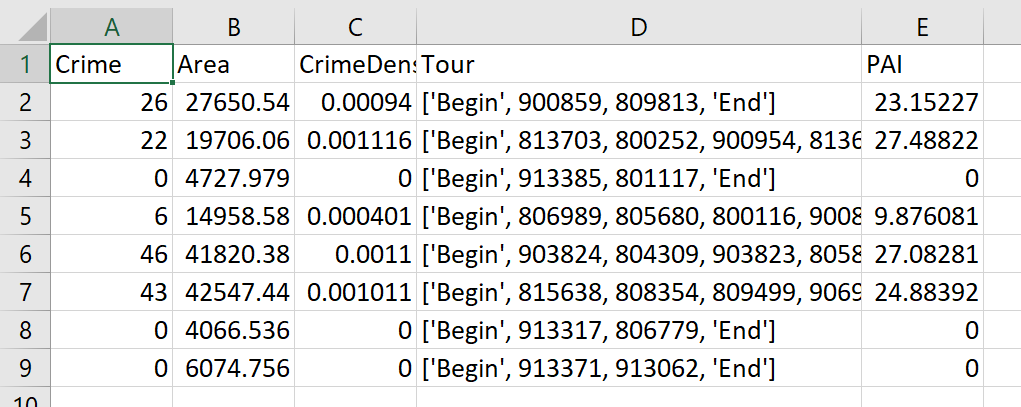You can see that it ends up being the tours have higher PAI values, but lower overall crime counts.

You may think, why does it not pick at least singlet areas with at least one crime? It ends up being that I weight areas here by their area (this formulation would be better with grid cells of equal area, so my objective function is technically `Sum [ D_ij * w_j *( CrimeDensity_j - DensityPenalty_j ) ]`, where w_j is the percent of the total area (so the denominator in the PAI calculation). So it ends up picking areas that are the tiniest areas, as they result in the smallest penalty to the objective function (w_j is tiny). I think this is OK though in the end – I rather know that some of the tours are worthless.

You can also see I get one subtour that is just under the PAI 10 threshold. Again possible here, but should be only slightly below in the worst case scenario. The way the objective function works is that it is pretty tricky to pick out subtours below that PAI value but still have a positive contribution to the overall objective function.

# Future Directions

The main thing I wish I could do with the current algorithm (but can’t the way the linear program is set up), is to have minimum and maximum tour area/length constraints. I think I can maybe do this by adapting this code (I’m not sure how to do the penalties/objectives though). So if others have ideas let me know!

I admit that this may be overkill, and maybe just doing more typical crime clustering algorithms may be sufficient. E.g. doing DBSCAN hot spots like I did here.

But this is my best attempt shake at the problem for now!

# An example of soft constraints in linear programming

Most of the prior examples of linear programming on my site use hard constraints. These are examples where I say to the model, “only give me results that strictly meet these criteria”, like “only select 40 cases to audit”, or “keep the finding rate over 50%”, etc.

There are alternative ways though to tell the model, “I want to select a finding rate over 50%, but still potentially consider those with lower finding rates”. One way to do that is via soft constraints, modifying the objective function directly to penalize (or favor) particular outcomes. For example, say you knew you could translate a 1% finding rate difference over 50% to a value of \$1000. So if our original model is:

``````Maximize Sum{D_i*Return_i}

Subject To
D_i element of (0,1) #decision variables are 0/1
Sum{D_i} = 100       #so select 100 cases``````

We would then place an additional penalty term that looks like this:

``````Maximize Sum{D_i*Return_i} + Sum{D_i*[(prob_i - 0.5)*1000]}

Subject To
D_i element of (0,1)
Sum{D_i} = 100``````

So instead of a subject to constraint that says we need to be over 50% finding rate, we added a second penalty term for solutions that have an under 50% finding rate. So here if the finding rate in the end is 49%, it takes a hit of \$1000 in the objective function. This example is also similar to a bi-objective function, here I just set an exact translation between finding rates and returns, but in practice often you don’t have that exact translation.

It just depends on your situation whether hard constraints or soft constraints make sense. Many situations you can swap one for the other, so different means same ends. For a good example of this, my allocating police resources paper on reducing disproportionate minority contact uses hard constraints (Wheeler, 2020), and George Mohler and colleagues have a very similar paper which uses soft constraints (Mohler et al., 2018). I imagine these will end up being very similar ends, although in that circumstance I prefer my hard constraint approach, as George’s you need to fiddle with the magnitude of the penalty term. Also I don’t tend to like changing the loss function for statistical/machine learning models, I just like changing what you do with the info after you have fit your model (Kleinberg et al., 2018).

Here I provide an example of where I think soft constraints make a bit of sense though. Imagine you have continuous predictive outputs, you need to make a binary yes/no decision among those options, but those predictive outputs also have a variance. An example of where this comes up is if you are making loan decisions, you want your portfolio to have a high return, but you also want to lower the variance of those returns as well.

For a simple example, imagine you are the lending institution and you have the choice between two scenarios:

• Scenario A: lend 1 person \$100,000 with an expected return of \$8,000, with a variance of \$4,000
• Scenario B: lend 2 people \$50,000, with an expected return of each for \$4,000 each, with a variance of \$1,000 for each loan

Since you expect to make the same amount of money under each scenario, option B is preferable if the loans are independent of each other (e.g. one going under does not cause the other to go under). In that case, variances are additive, so the total variance of option B is \$2,000, so has much less volatility than does the A scenario.

(Sorry to my criminology friends, this example is generic but I strain to find a criminal justice example to apply it to. It would not be crazy that you have low volatility vs high volatility hot spots. So you may want to choose a consistent hot spot as oppossed to a fleeting one for an intervention. But I don’t think that will happen in practice quite like that. Choosing among expensive high risk/reward vs inexpensive treatment regimes low risk/reward in corrections settings may also make sense, but that is crazy pie in the sky technocratic given the current state of affairs as well.)

# Example with Lending Club Data

So to illustrate an example with actual data, I’ve provide Python code fitting a predictive model to Lending Club data on loans. (I got the original dataset from Kaggle.) I am just going to highlight some key points here in the blog post. You will need to go to the code to see everything.

First, I’ve been introduced to this dataset as predicting a binary default/no-default. I have code doing that in the code snippet as well, and it performed OK. But it was very uncalibrated as to whether my portfolio made money – so even though the default estimates were pretty well spot on, my portfolios did not make much money. This is because people who default pay back some loans, and also quite a few people in the dataset pay back the loans fast, so the lenders don’t make as much as interest as you would expect at the start of the loan.

So I cut out the middle man and just estimated a random forest model predicting the actual money one made on the loan. I only kept cases that are either `'Fully Paid','Charged Off', 'Default'`, so I don’t model loans that are still ongoing. I end up modeling then the value `total_pymnt - loan_amnt`. You can look into the code to see the variables I included in the model, but one of the neat things about regression random forests is that you can not only get the mean prediction, you can also look at the variance over all the trees. See below a function to do that (in the 01 py file):

``````###############################################################
#Fit random forest model
model = RandomForestRegressor(n_estimators=1000,
random_state=10,
min_samples_leaf=100)
model.fit(train[x_vars], train[y_var])

#Check the predicted vs actual on the test set
y_pred = model.predict(test[x_vars]) #predicted mean
test['y_pred'] = y_pred

#I want an estimate of the variance
def tree_var(X, rf_mod):
per_tree_pred = [pd.Series(tree.predict(X), index=X.index) for tree in rf_mod.estimators_]
pd_res = pd.concat(per_tree_pred, axis=1)
pd_var = pd_res.var(axis=1)
return pd_var

test['y_var'] = tree_var(X=test[x_vars], rf_mod=model)
###############################################################``````

And that predicted value and variance are then what I feed into my subsequent linear programming problem (in the 02 py file). The model in some more text is:

``````Maximize Sum{D_i*(prediction - lambda*variance)}

Subject To:
D element of (0,1)                #decision variables
Sum{D_i*loan_amount_i} <= 300000  #only have so much \$ to loan, so no leveraging
``````

Where lambda is the tuner – higher variances will get higher penalties. So going back to our two loan example, if lambda = 1, scenario A it would be `8000 - 1*4000 = 4000`, and scenario B would be `8000 - 1*2000 = 6000`, so that penalty would choose scenario B over A. Whereas without the penalty the two scenarios are exactly the same.

Since the lambda value is arbitrary, I illustrate the approach selecting portfolios of loans that are a total of \$300,000 (I divided the loans by \$1000 to make the numbers a bit easier to view). This is a totally held out sample of around 5k loans. So you can see my first model (with no constraints):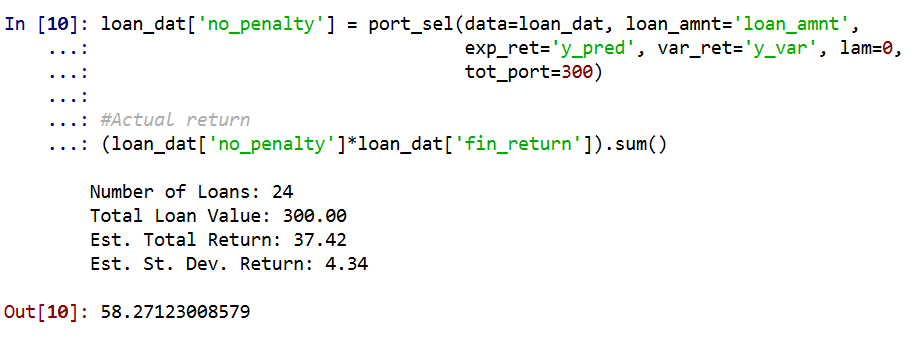And my second model with a higher lambda value of 1 selects more (smaller) loans, and reduces the variance. You can see since we have the actual outcomes, I can show that both portfolios turned a profit, each above what I predicted. But the standard deviation for second portfolio is cut not quite in half.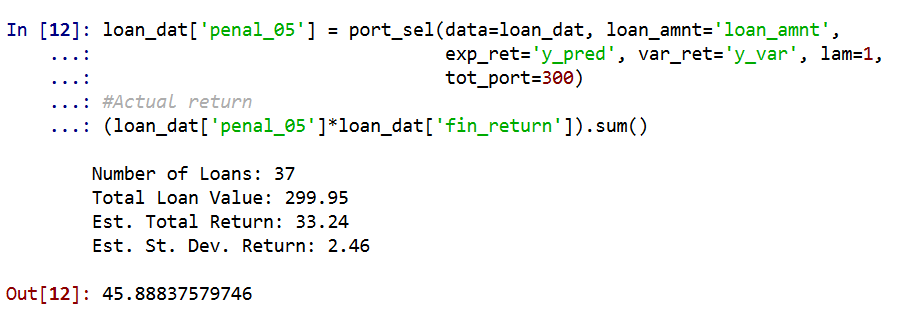So you can see in that one selection it worked out OK, but this does not verify that my variance estimates are correct (they are no doubt too small, as you can see the actual returns are way higher than I predicted).

To test them out though I do a simulation. I draw 1000 cases out of those 5000, and then again pick \$300k in loans. I do that process 1000 times under a set of different lambda penalty terms, `[0, 0.5, 1.0, 2.0, 3.0]`. For those simulations, here is the overall distribution of the returns under different penalty terms for the variance.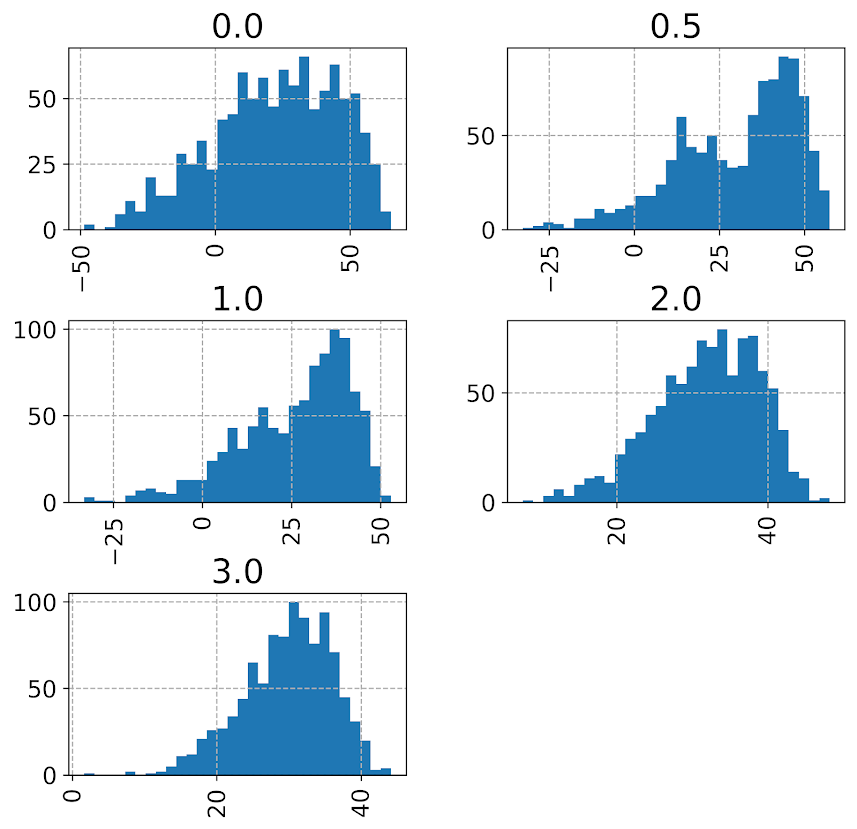Note that those histograms have different X axes. It is easier to see the moments of the spread in a boxplot: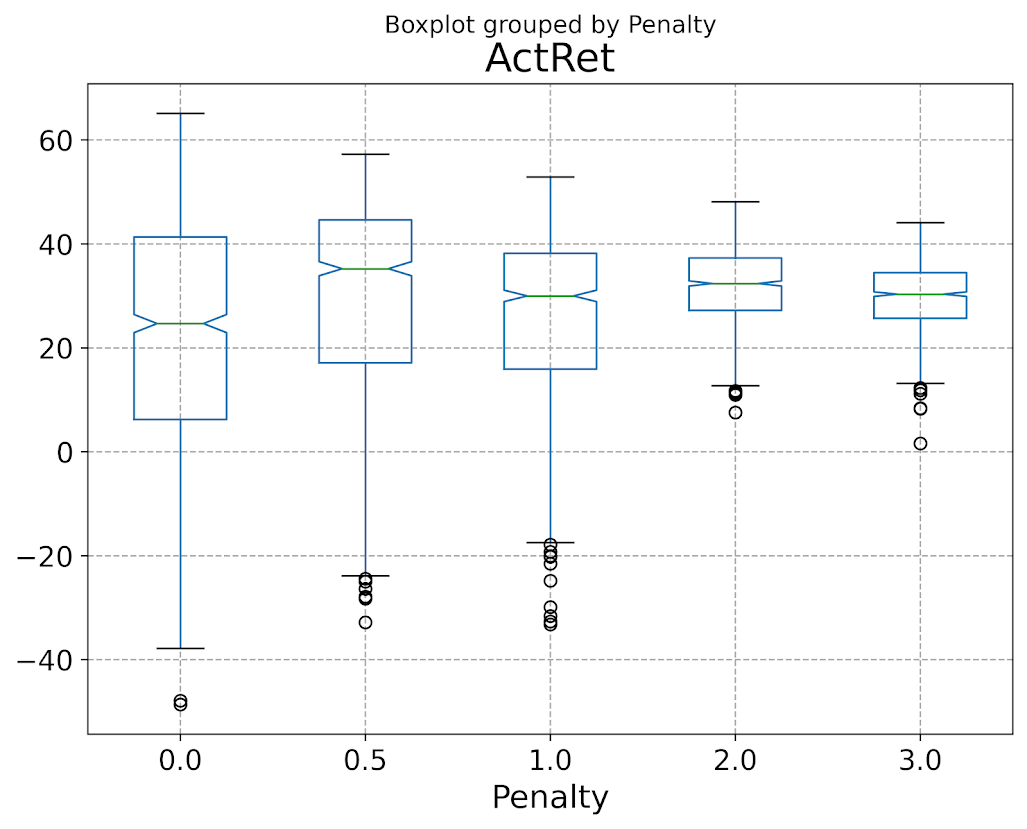So here you can see each scenario has pretty near the same median return (somewhere around \$30k), but the penalties reduce the variance. The higher penalties end up selecting portfolio’s that always at least make money, whereas the lower penalty terms you do end up losing money in some scenarios.

Unfortunately I did not beat the market in my simple weekend experimentation, so don’t sink a bunch of money into Lending club based on this! The average returns starting from \$300k are something like an annualized rate of return of around 3% (over 3 years) for the smaller simulation pick from 1000. These include loans of 60 months as well though. So even though my linear program with the penalty term did a good job of reducing the risk, this isn’t good enough returns for me to put a bunch of my money into Lending Club.

But there is no doubt improvements both to the modeling as well as the portfolio selection. For modeling I would be tempted to try out a discrete time survival model for payments over time, but that would be more work than I could do in a weekend. (Also I only incorporated the easy continuous variables here I could prepare in just a few minutes, so maybe more feature engineering would boost my results.)

I could also adapt the linear program to take into account covariance between the loans, but not sure how to estimate them (a multi-level model perhaps?). You also may want to do some sort of conditional value at risk approach in the linear program, say instead of piping in the variance from random forests, count up how often you lose money and put that as a penalty or constraint on the system.

# An intro to linear programming for criminologists

Erik Alda made the point the other day on twitter that we are one of the few crim folks that do anything related to linear programming. I think it is crazy useful – much more so than say teaching myself some new regression technique or a programming language.

I don’t quite remember the motivation to learn it. I think I kept seeing repeated applications in papers I read, but was also totally baffled by it; I did not understand peoples notation for it at all. In retrospect that was because it is not statistics. You are optimizing a function by estimating some parameters (there is nothing stochastic about it, so there is no statistical inference). So it is more like finding the min/max of a function in calculus.

I think the best way to think about linear programming is in terms of decision analysis. We have a set of options among which we need to choose some action. So we make the choices that either maximize or minimize some objective, but also take into account constraints on the decisions we can make.

For social scientists here is an example that hopefully illustrates the difference between statistics and linear programming. Say we are interested in conducting a hot spots policing randomized experiment. So we define our top 20 crime hot spots in the city, and randomly assign 10 of them to receive the hot spots treatment. Linear programming is basically the obverse of this, given our 20 hot spot areas, which are the best 10 locations to choose for our intervention.

This problem as stated you might be thinking is trivial – just rank each of the 20 hot spots by the total number of crimes, and then choose the top 10. Where linear programming really helps though is if you have constraints on the final choices you make. Say you did not want to choose hot spots that are within 1 mile of each other (to spread out the hot spot interventions throughout the city). There is no simple way to sort your hot spots to obey that constraint, but you can encode that in the linear program and have the computer solve it quite easily.

There is no shortage of ways you could expand the complexity of this example hot spot decision analysis. Say you had two different types of hot spot treatments, and that they had different efficacy in different areas (one was good for property crime, and the other was better for violent crime). You might think of this as doing two separate decision analyses, where a constraint is that an area can only be assigned one of the two interventions.

Here I will provide some code examples in python using the pulp library to illustrate some more examples using data you can see in action, as well as different ways to think about linear programming problems in practice. (Technically the examples I give are all mixed integer linear programs, as the decision variables are binary 0/1.)

# Formulating Objectives and Constraints

For this example I will be simulating data, but imagine a case you are an analyst for the IRS, and you want to determine which business tax returns to audit. We want to audit cases that both have a high probability of being fraudulent, as well as cases in which the total amount of the underpayment is large. (If you want a more typical criminology example, imagine assigning criminal cases to detectives, some cases have more costs, e.g. homicide vs burglary, and some cases have different probabilities of being solvable. This type of decision problem is very common in my experience – pretty much any time you have to make a binary choice, and those choices have variable costs/benefits.)

First I start off by simulating some data (the only libraries we need are numpy and pulp). So I simulate 1000 business tax returns, which have an estimate of the probability they are fraud, `prob_fraud`, and an estimate of the amount they underpayed, `underpay_est`.

``````import numpy as np
import pulp

###########################################################
#Simulate data for costs and probabilities

np.random.seed(10)
total_cases = 1000
underpay_est = np.random.uniform(1000,100000,total_cases)
prob_fraud = np.random.uniform(0,1,total_cases)
exp_return = prob_fraud*underpay_est

###########################################################``````

The objective we will be maximizing then is the expected return of auditing a tax return, `exp_return`, which is simply the multiplication of the probability of fraud multiplied by the amount of the underpayment. For a simple example, say we have a case where fraud is estimated to be 50%, and the estimate of the underpayment amount is \$10,000. So our expected return for auditing that case is \$5,000.

We need these two estimates external to our linear programming problem, and they themselves can be informed by predictive models (or simpler estimates, e.g. underpayment is proportional ~30% of deductions or something like that).

Now we have all we need to set up our linear programming problem. I am going to choose 100 cases out of these 1000 to audit. Hopefully that code is documented enough to see creating the decision variables (each tax return either gets a 1 if it is chosen, or a 0 if it is not), the original objective function that we are maximizing, and the results.

``````#Setting up the problem
case_index = list(range(total_cases))
tot_audit = 100

####################################
#Basic Problem
P = pulp.LpProblem("Choosing Cases to Audit", pulp.LpMaximize)
D = pulp.LpVariable.dicts("Decision Variable", [i for i in case_index], lowBound=0, upBound=1, cat=pulp.LpInteger)
#Objective Function
P += pulp.lpSum( D[i]*exp_return[i] for i in case_index)
#Constraint on total number of cases audited
P += pulp.lpSum( D[i] for i in case_index ) == tot_audit
#Solve the problem
P.solve()
#Get the decision variables
dec_list = [D[i].varValue for i in case_index]
dec_np = np.asarray(dec_list)
#Expected return
print( (dec_np * exp_return).sum() )
#Should be the same
print( pulp.value(P.objective) )
#Hit rate of cases
print( (dec_np * prob_fraud).sum()/tot_audit )
####################################``````

If you are following along in python, you will see that the total expected return is `7,287,915`, and the estimated hit rate (or clearance rate) of the audits is around `0.88`.

This example would be no different if we just chose the top 100 cases based on the expected return. Say that you thought the hit rate though of 88% was too low. You will choose cases that are big dollar amounts, but not necessarily a very high probability. So you may say I want my clearance rate to be over 90% overall. That is a simple constraint to add into the above model.

``````####################################
#Updating the problem to constrain on the hit rate
#Above a particular threshold
hit_rate = 0.9
P += pulp.lpSum( D[i]*prob_fraud[i] for i in case_index ) >= hit_rate*tot_audit
P.solve()
#Get the decision variables
dec_list = [D[i].varValue for i in case_index]
dec_np = np.asarray(dec_list)
#Expected return is slightly lower than before
print( pulp.value(P.objective) )
#Hit rate of cases
print( (dec_np * prob_fraud).sum()/tot_audit )
####################################``````

So now the total expected return is lower than without the constraint, `7,229,140` (so a reduction of about \$60k), but our expected hit rate is just above 90%.

You may be thinking, “why not just eliminate cases with a probability of lower than 90%”, and then amongst those left over select the highest expected return. That meets your constraints, but has a lower expected return than this program! Think of this program as more tit-for-tat. High expected return / lower probability audits can still be selected with this model, but you need to balance them out with some high probability cases in response to tip the scales to meet the overall hit rate objective.

# Trade-Offs and the Frontier Curve

So you may be thinking, ok the trade-off to get a 90% clearance was not too bad in terms of total extra taxes collected. So why not set the constraint to 95%. When you create constraints, they always lower the objective function (lower or equal to be more precise). The question then becomes quantifying that trade off.

You can subsequently vary the hit rate constraint, and see how much it changes the total expected return. Here is an example of doing that, each model only takes around a second to complete.

``````###########################################################
#Drawing the trade-off in hit rate vs expected return

hit_rate = np.linspace(0.85, 0.95, 30)
total_return = []

#Function to estimate the model
def const_hit_rate(er, prob, tot_n, hr):
c_index = range(len(er))
Prob = pulp.LpProblem("Choosing Cases to Audit", pulp.LpMaximize)
Dec = pulp.LpVariable.dicts("Decision Variable", [i for i in c_index], lowBound=0, upBound=1, cat=pulp.LpInteger)
Prob += pulp.lpSum( Dec[i]*er[i] for i in c_index)
Prob += pulp.lpSum( Dec[i] for i in c_index ) == tot_n
Prob += pulp.lpSum( Dec[i]*prob[i] for i in c_index ) >= hr*tot_n
Prob.solve()
dec_li = [Dec[i].varValue for i in c_index]
dec_np = np.asarray(dec_li)
return pulp.value(Prob.objective), dec_np

for h in hit_rate:
print(f'Estimating hit rate {h}')
obj, dec_res = const_hit_rate(exp_return, prob_fraud, 100, h)
total_return.append(obj)

###########################################################``````

For this simulated data example, there end up being pretty severe trade-offs in the total return after you get above 91% hit rates, so from this it may not be worth the trade-off to get a much higher hit rate in practice. Just depends on how much you are willing to trade-off one for the other.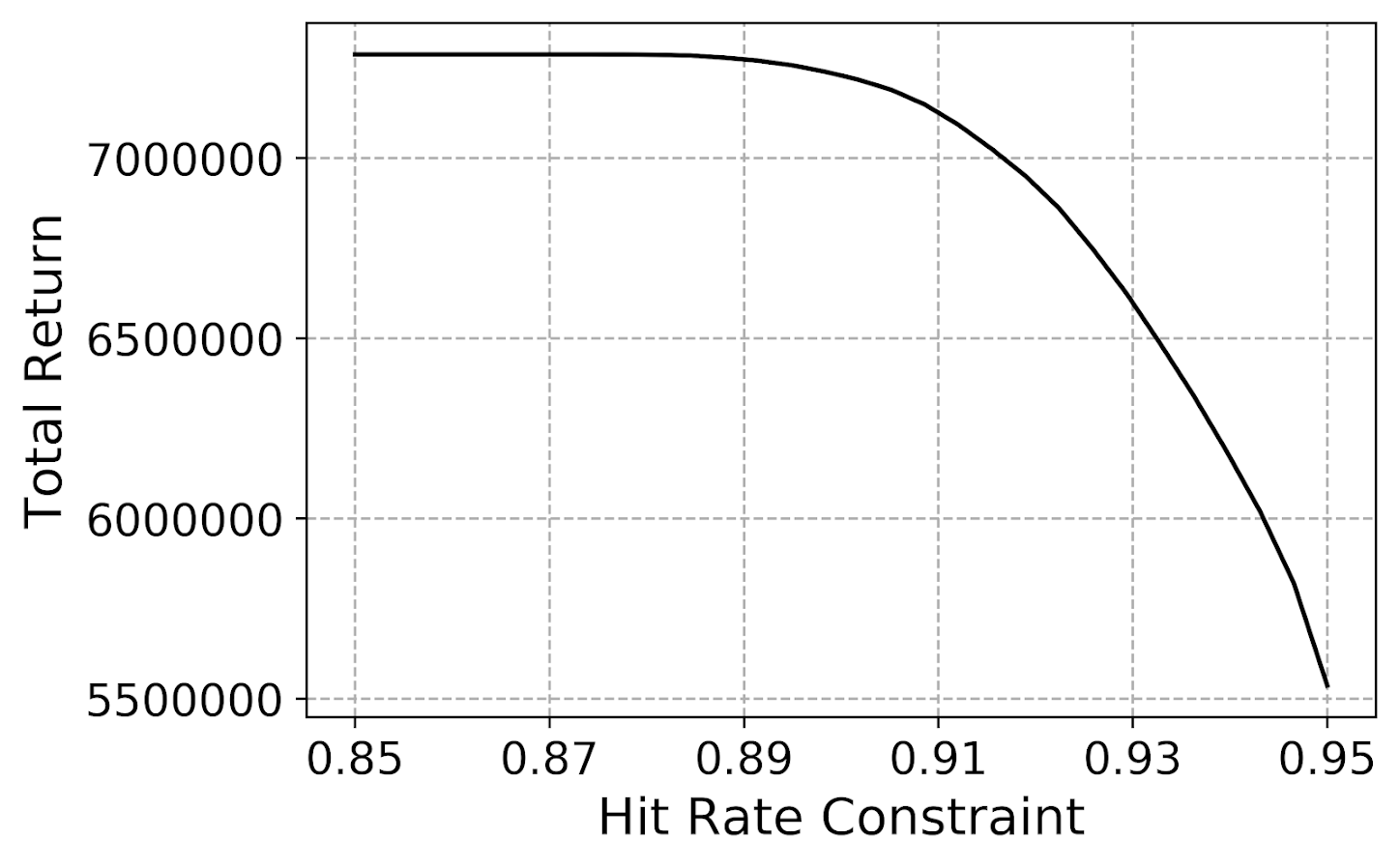There are other ways to formulate this trade off (via bi-objective functions/soft-constraints, or weighted ranking schemes), but the blog post is long enough as is!

# Other Potential Applications

So in terms of my work, I have examples of using linear programs to make spatial location decisions, encode fairness constraints into predictive policing, and allocate treatment assignment with network spillovers.

Erik Alda and Joseph Ferrandino have conducted frontier analysis of different criminal justice organizations, which is based on estimating the frontier curve above from data (instead of a pre-specified objective function).

That is about it for criminologists that I know of, but there are plenty of applications towards criminal justice topics using linear programming (or related concepts). It is most popular among operations researchers, of which Laura Albert is one of my favorites. (Criminal Justice as a field might not exist for Albert Blumstein, who was also a very influential operations researcher.)

One of the things that makes this different from more traditional quantitative work in the social sciences is that again it is not statistics – we are not testing hypotheses. The contribution is simply formulating the decision problem in a tractable way that can be solved, and the drawing of the trade-offs I showed above.

It is one of the ways I really like it though – unlike saying how your regression model can be used to inform decisions, this much more explicitly shows the utility of the results of those models in some practice.